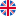English

### Functions and scripts (Formula field)

How to use the Minimum/Maximum, Ceil up/Ceil down (round) functions and Ruby scripts

## Minimum/Maximum functions

Let's see how the functions work, using the product table as an example.

This table contains the following columns:
Brand name and model;
Image;
Price for June, July and August.

The task is to show the lowest price for all three months.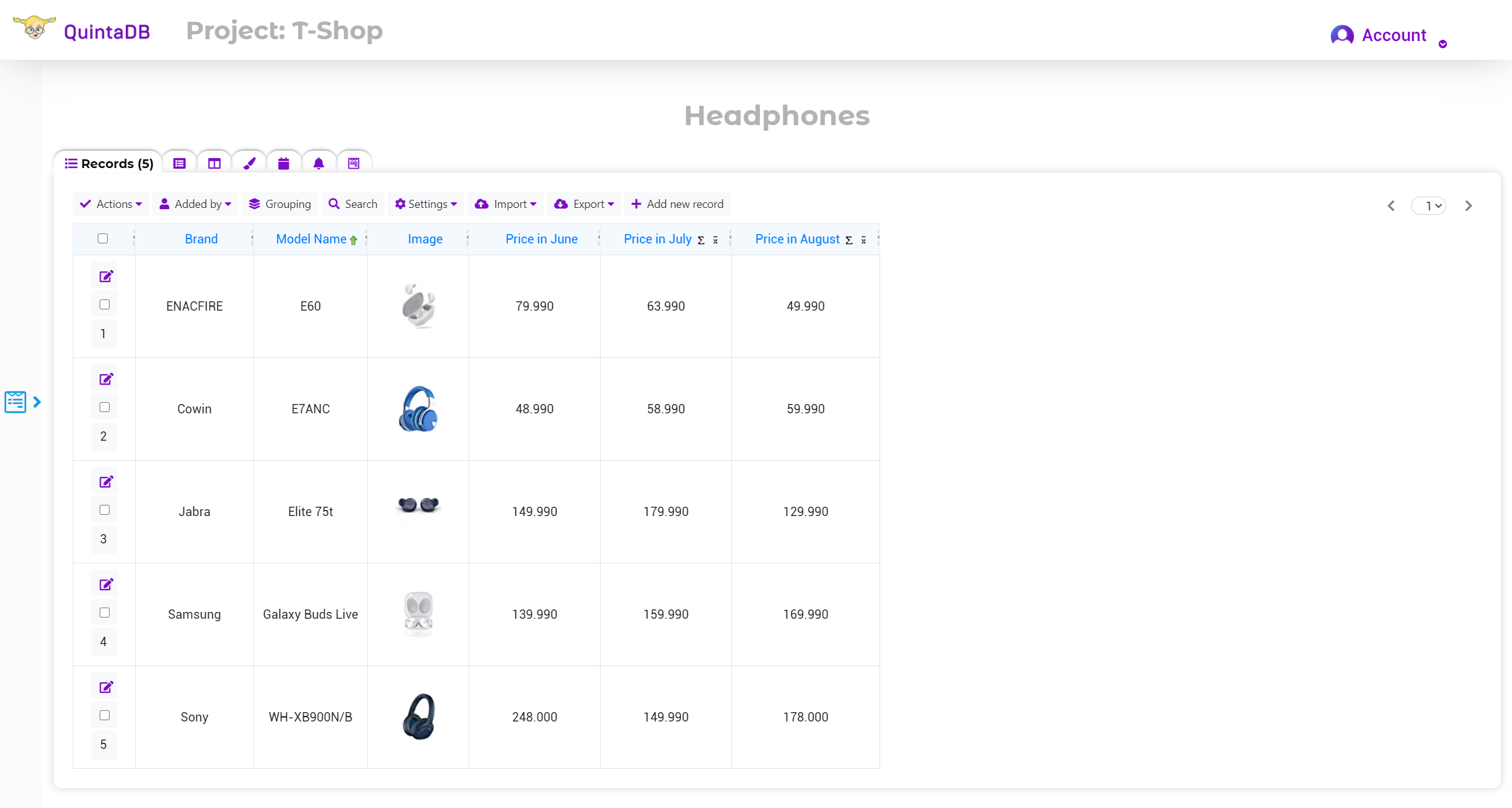The first step is to add the Formula field to the form.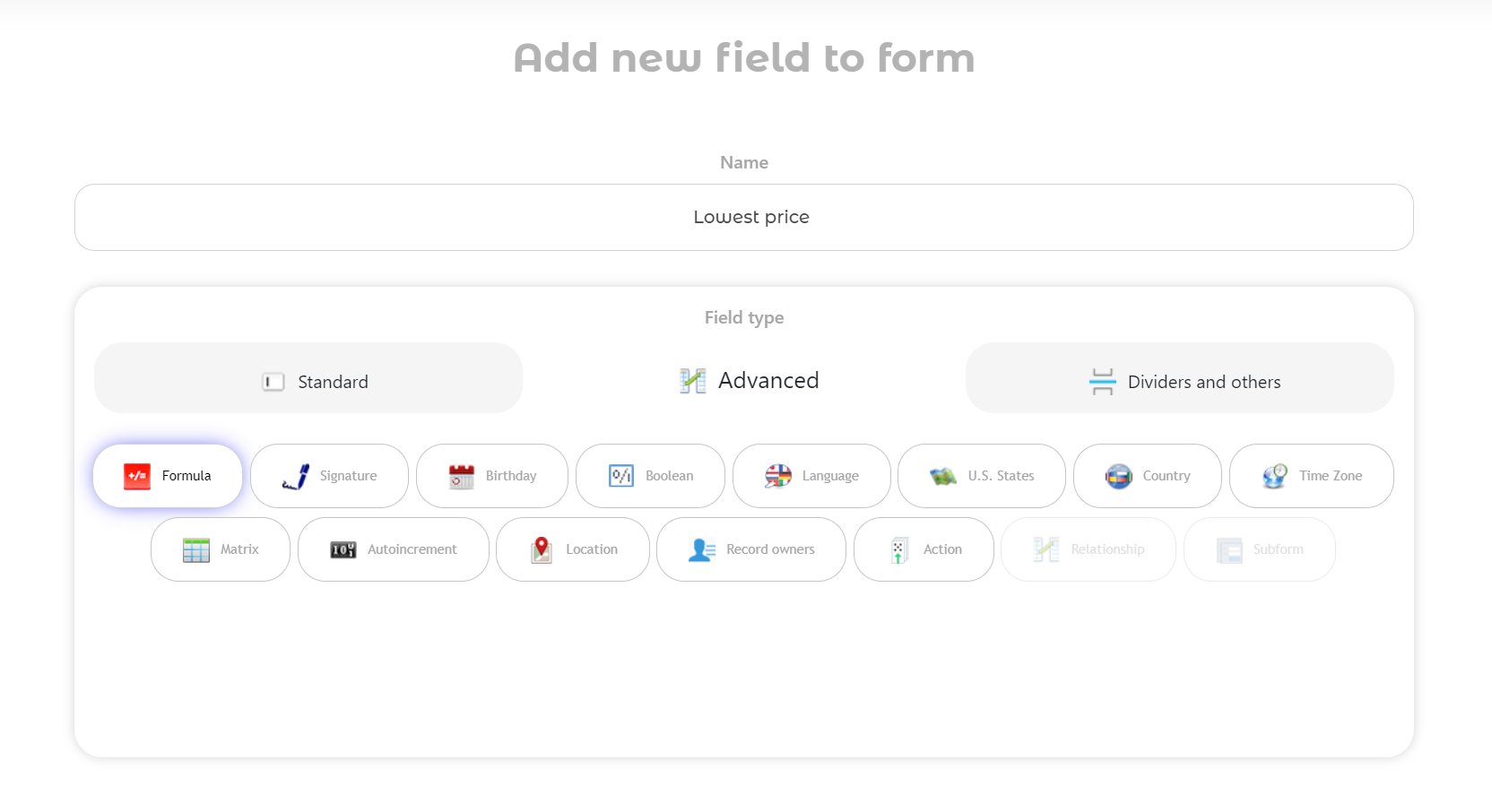Select "Function" in the first drop-down list:Select "Minimum" in the second drop-down list: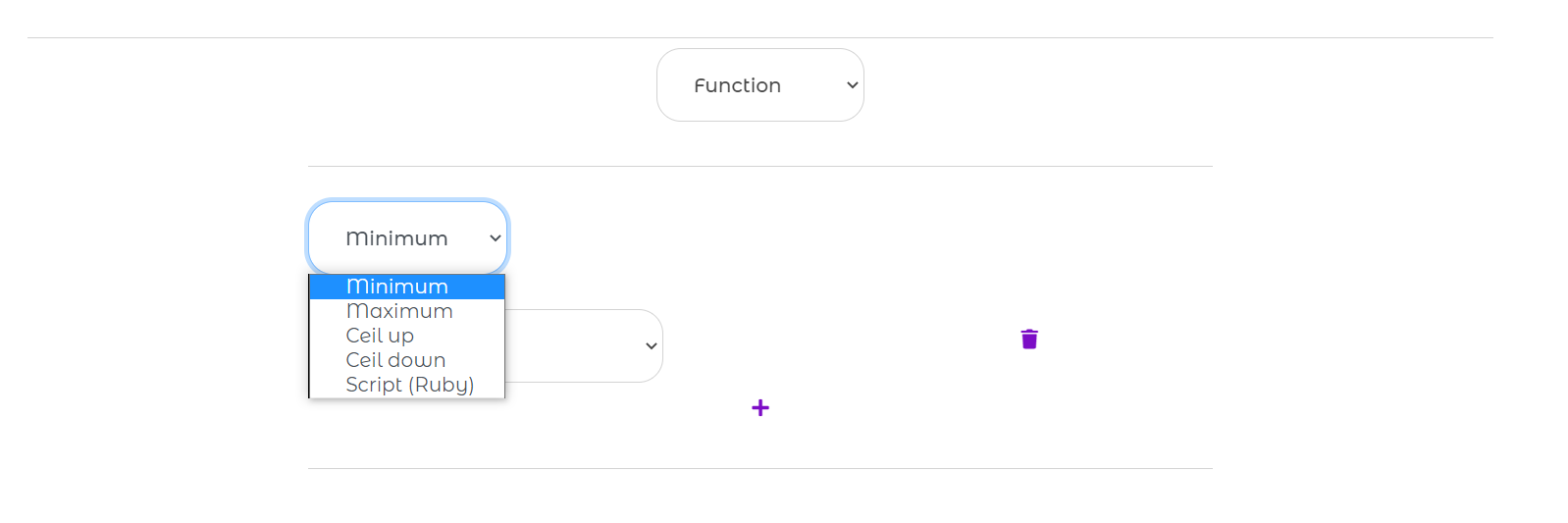Next, you need to select the fields for comparison. In this case, these are three price fields.
And click on the "Create" button.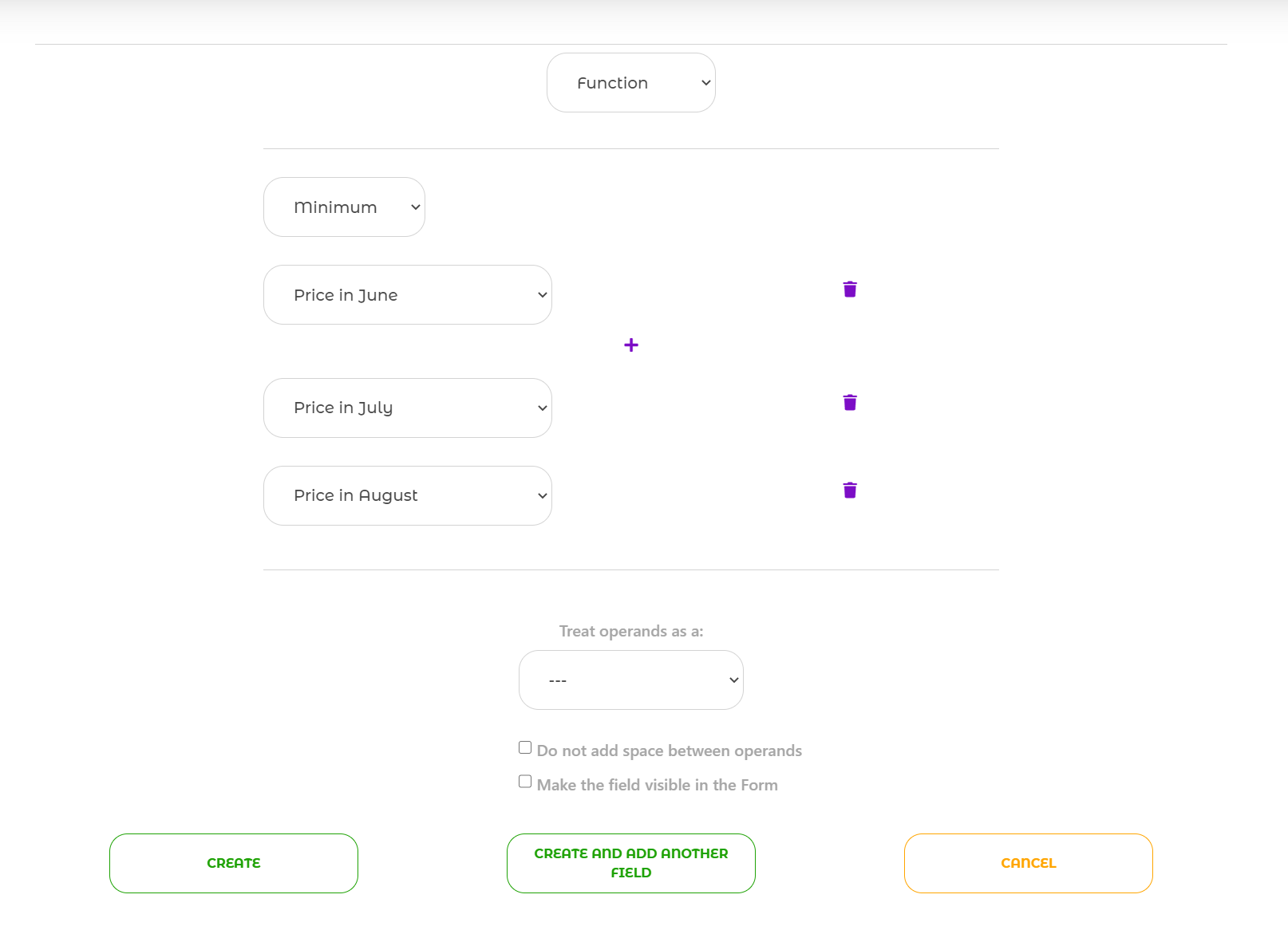Now the field "Lowest price" has appeared in our table, which compares the three values in the record and shows the minimum of them: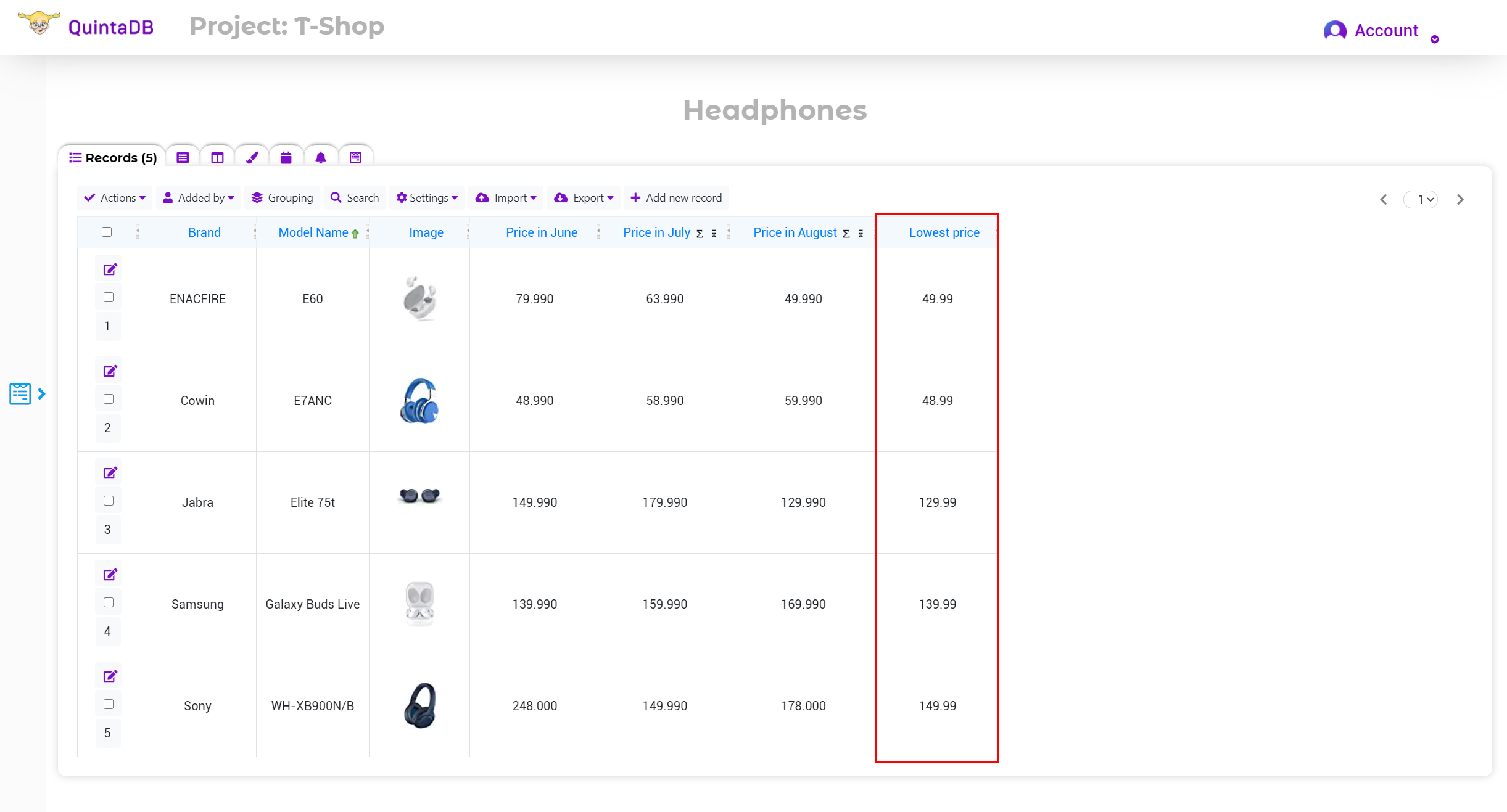The "Maximum" function works exactly the opposite.

## Ceil up/down functions

Consider the "Ceil up" and "Ceil down" functions. Here is a table with clients and their orders.

The task is to round the value in the "Payable amount" column.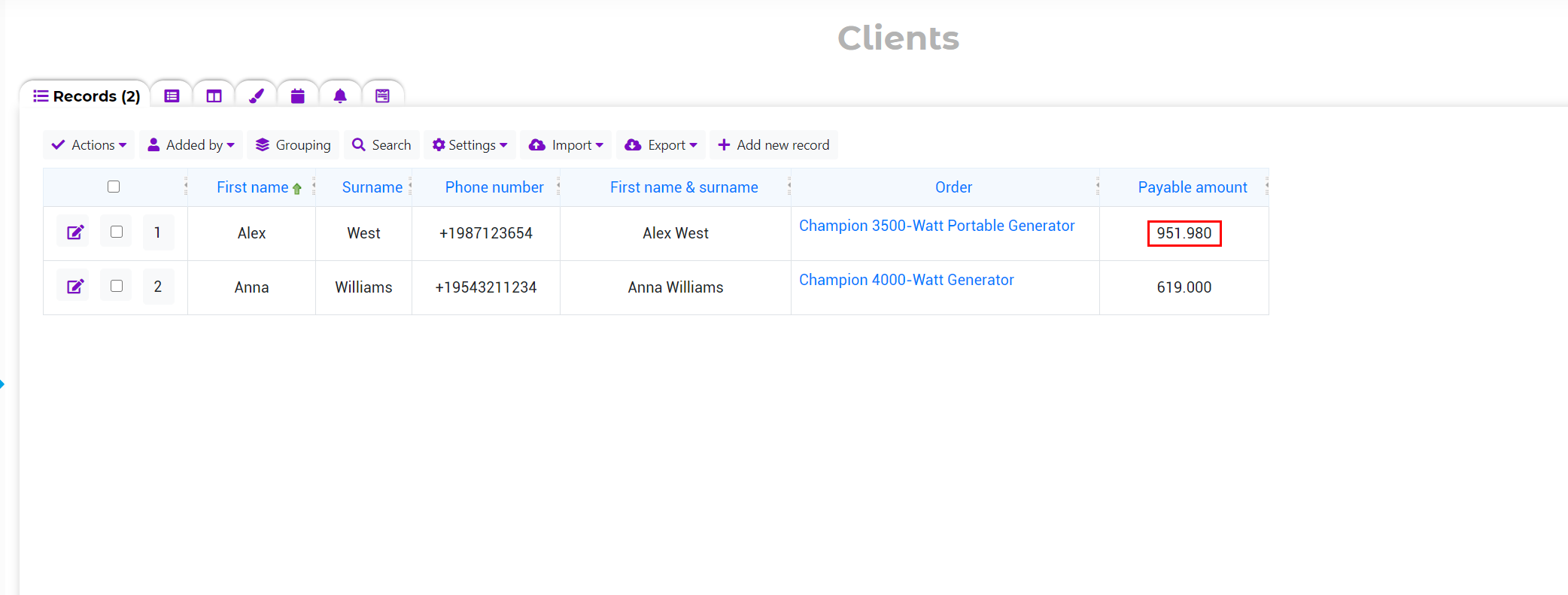Create a new Formula field and select the function "Ceil up" (or "Ceil down");

Next, you need to select the required field from the dropdown list and specify the significance.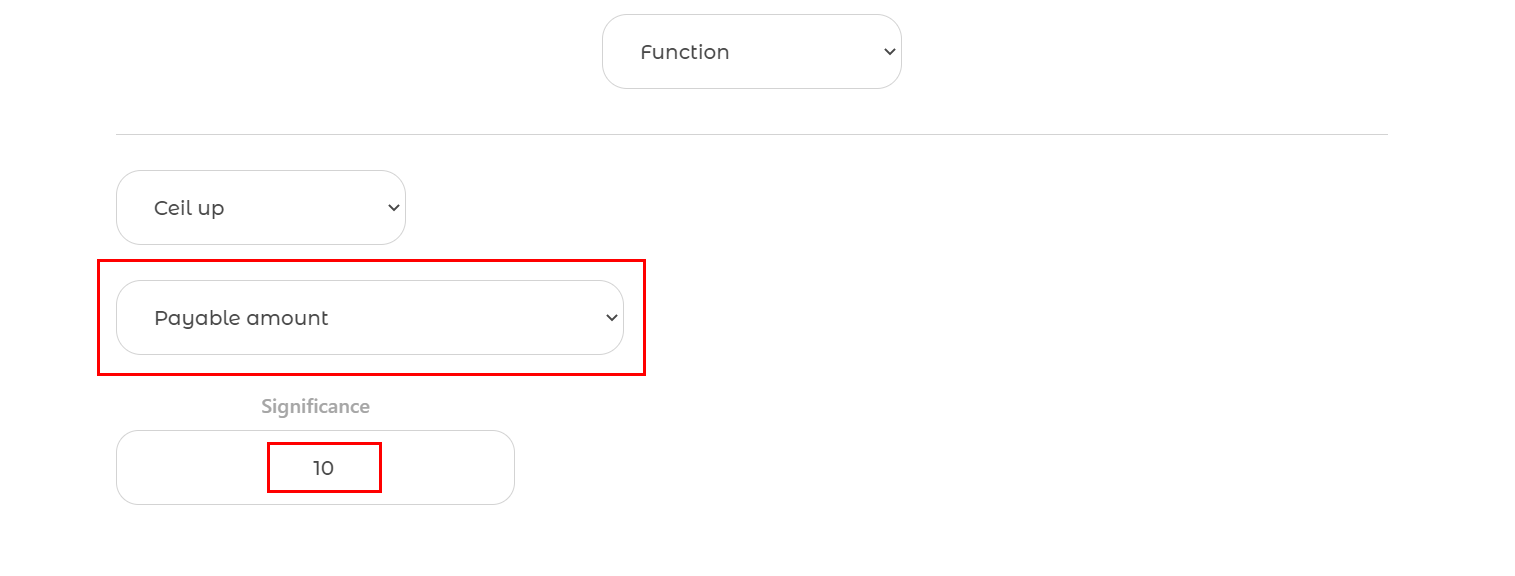As a result, we get a column with a rounded amount: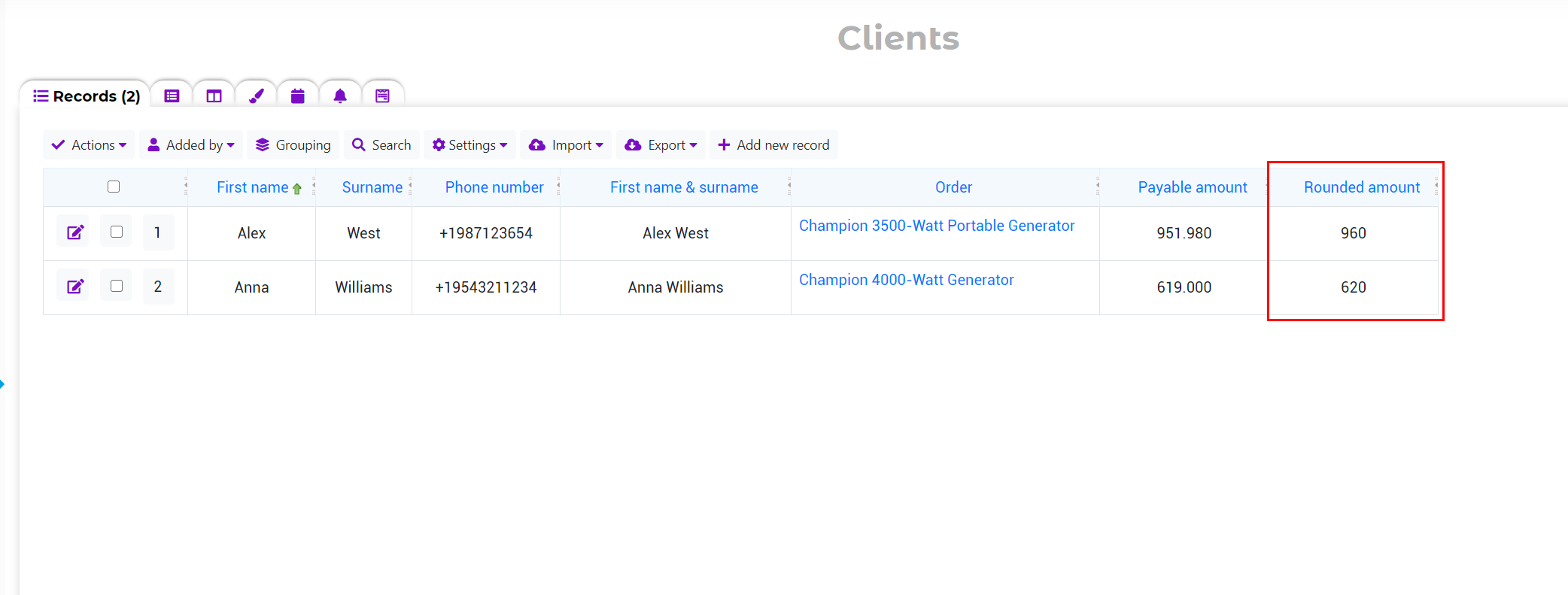## Scripts (Ruby)

Scripts allow you to perform logical and arithmetic operations.

We'll use the "Summer sales" table as an example. This table contains a column with a managers, their work duration and the sum of sales for the summer.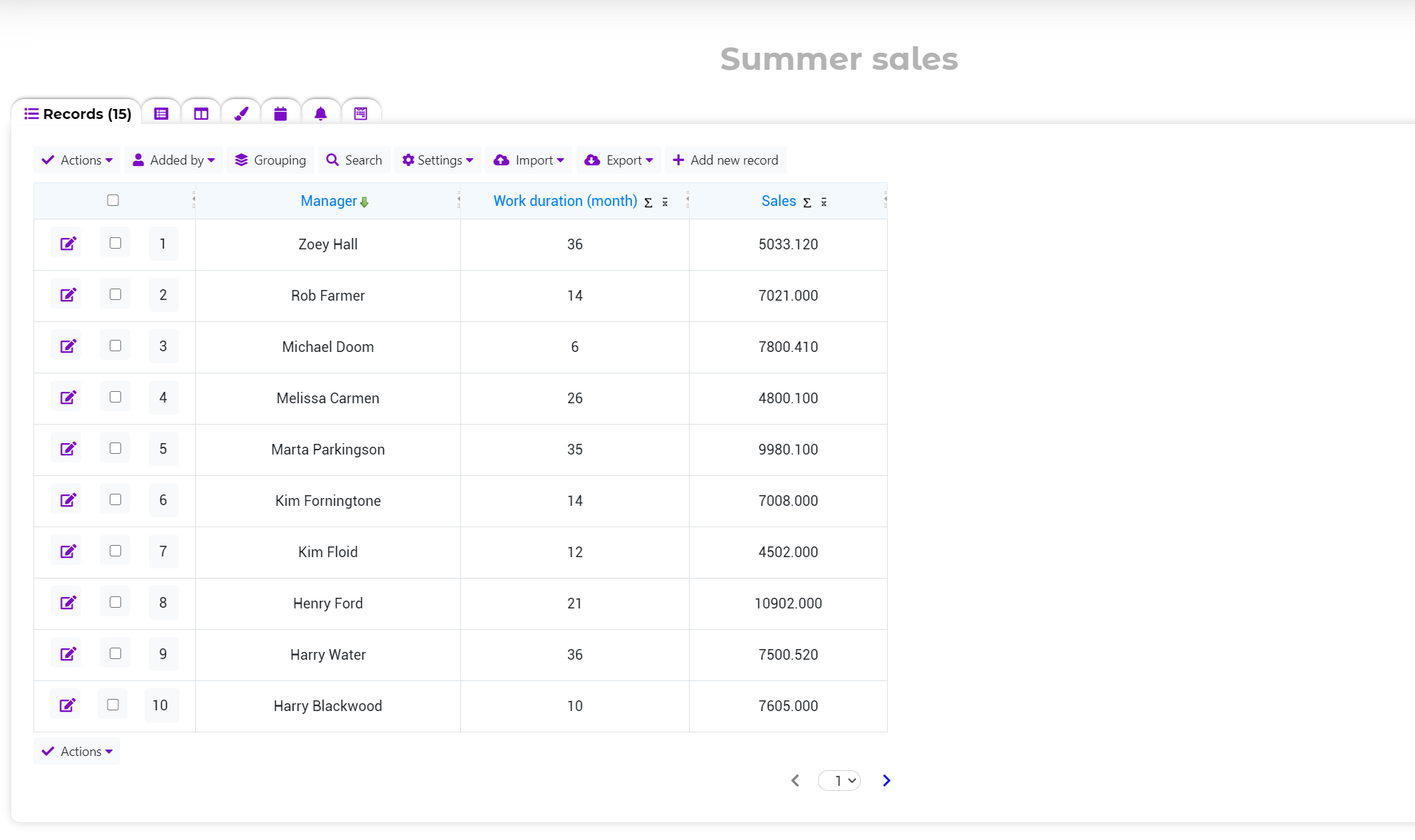Let's create a new Formula field, select the Function option and the Script (Ruby) item in the following list.

You can use HTML field names as operands.

Click on the link under the function field "How to insert record data":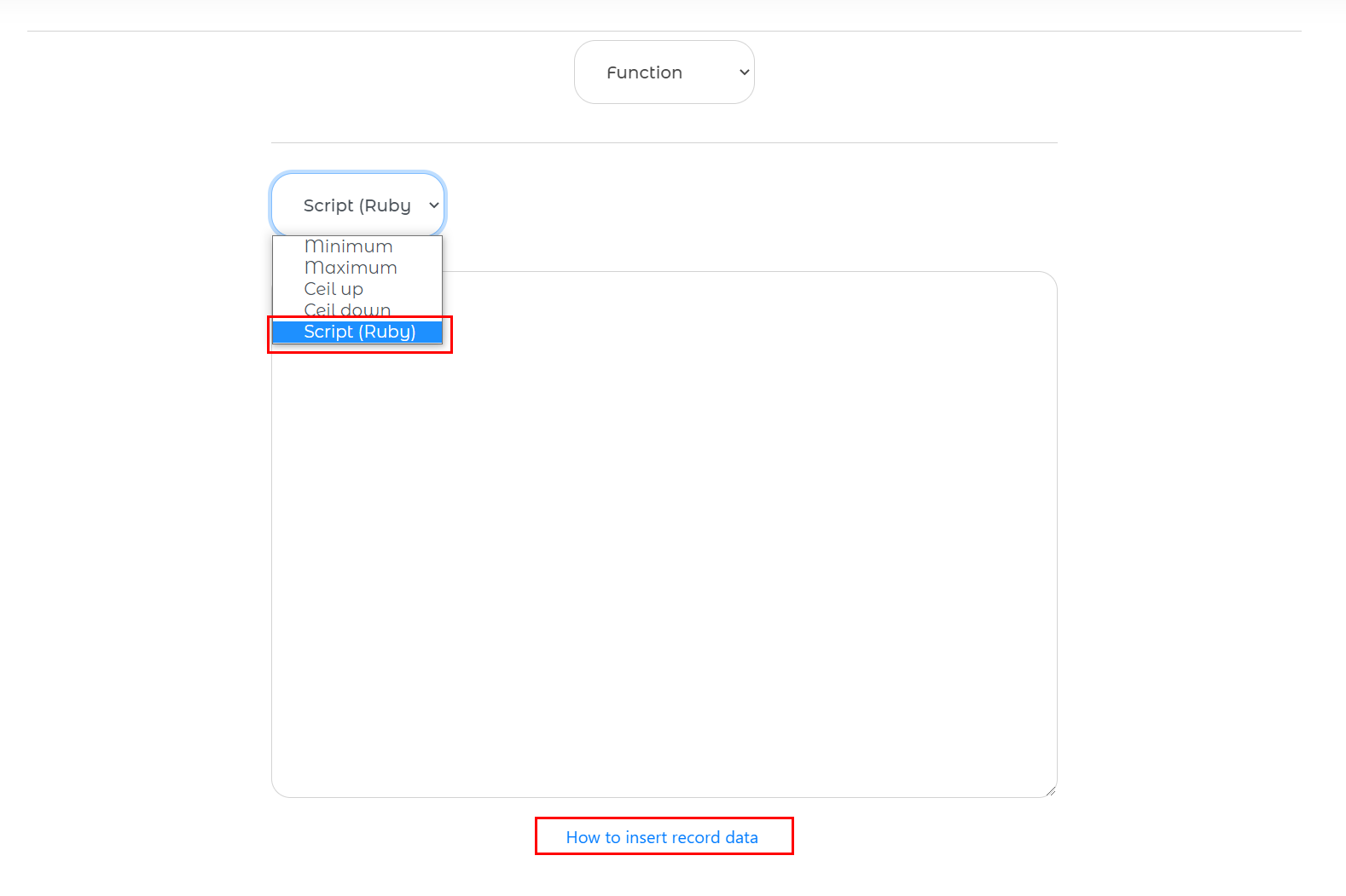A list of available fields will appear. Select a field from the list and copy HTML name.: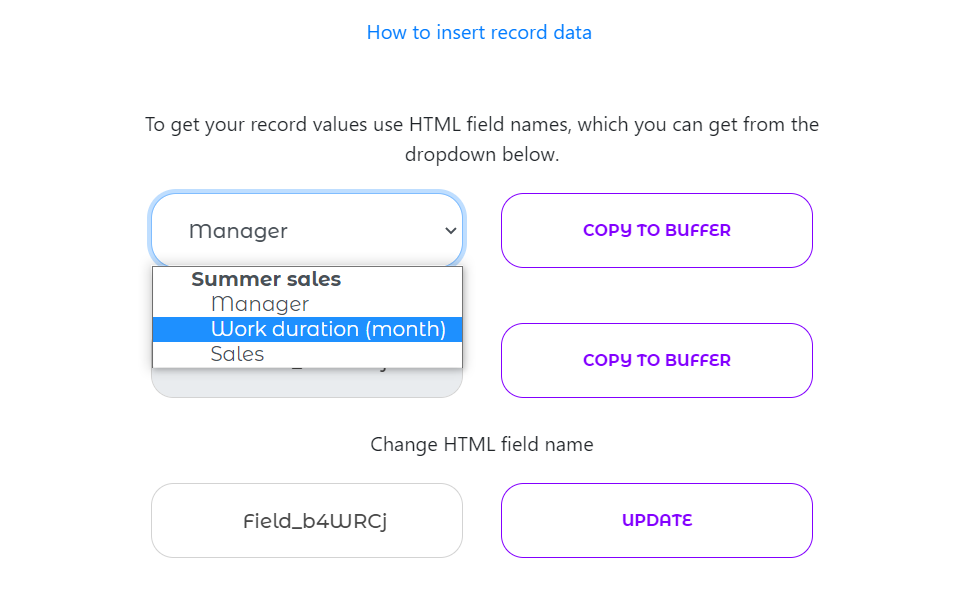Also, you can change the HTML field name right here. It is enough to enter a new name and click on the "Update" button: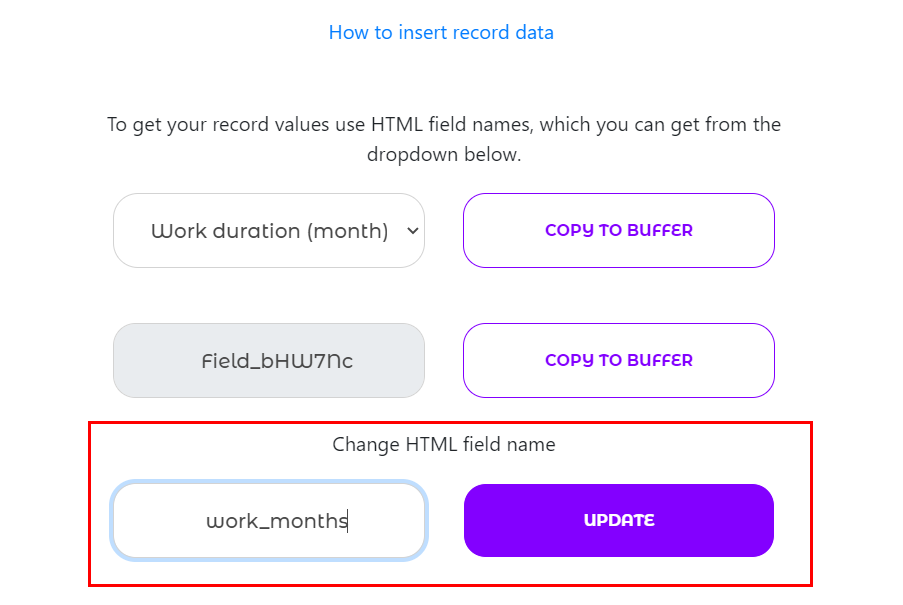The following Arithmetic and Conditional operators are available:

(-) subtraction

(*) multiplication

(/) division

(>) true, if the left operand is greater than the right

(<) true, if left operand is less than right

(%) modulo division

(==) true, if the values of the two operands are the same.

Conditional operators:

(if) using to test if a condition is true. Condition values false and nil are false, while all others will be True.

(||) using to test multiple conditions.

Let's go back, for example. The task is to calculate the bonus for managers.

Assume the bonus ratio depends on several factors. The first one is work experience in the company.

Let's create the following script:

if work_months > 12

300

This means, if an employee has worked in the company for more than 12 months, he will receive a bonus of  300 \$.

Let's create another condition. If the manager made more than 7000 sales, then he will receive 150 \$ + 25%.

The script should look like this:

elsif sales > 7000
(150*0.25)+150

In all other cases, the bonus will be 150 \$

else
150

It is necessary to specify end, for the script's correctly work.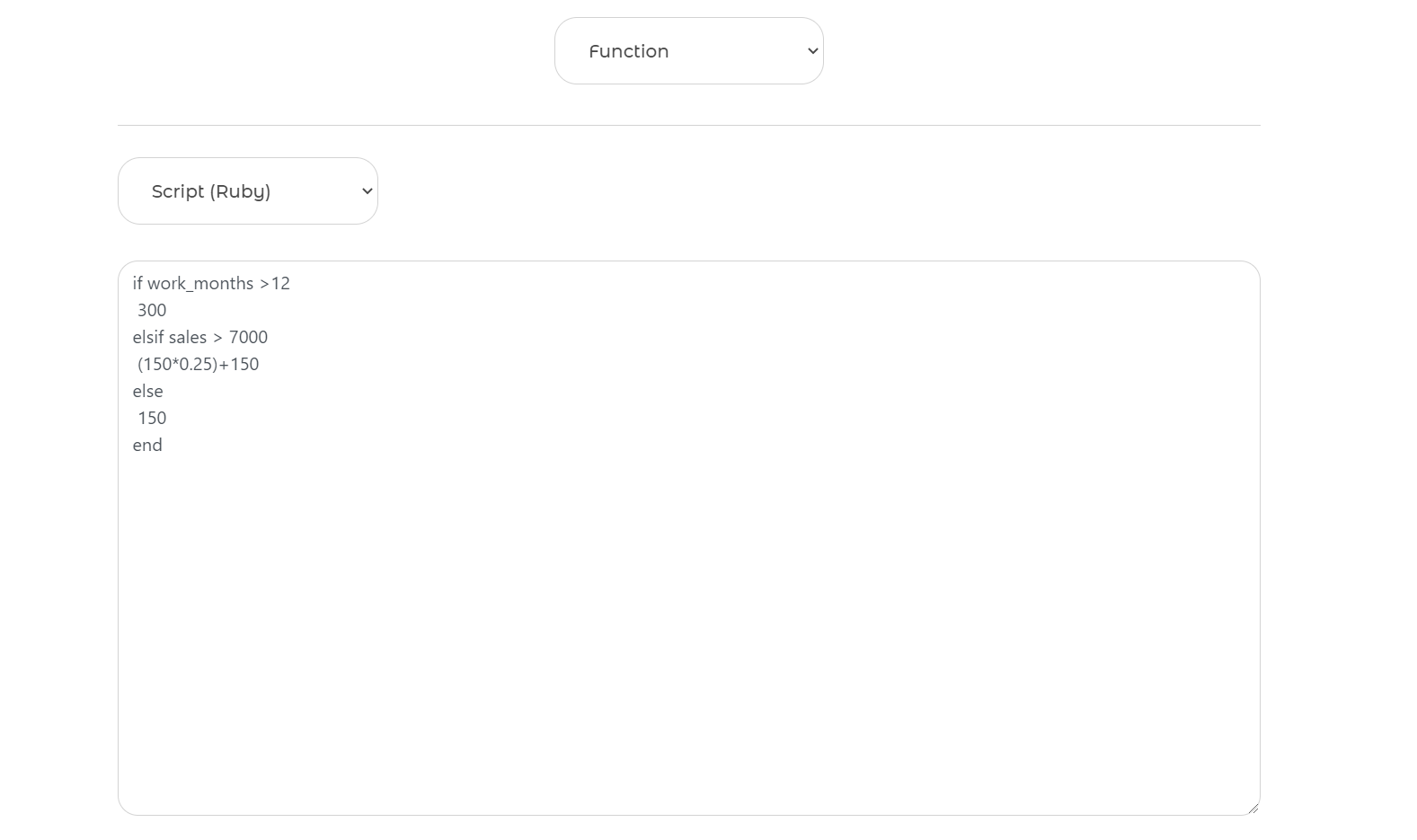Now we can calculate the bonus for each manager: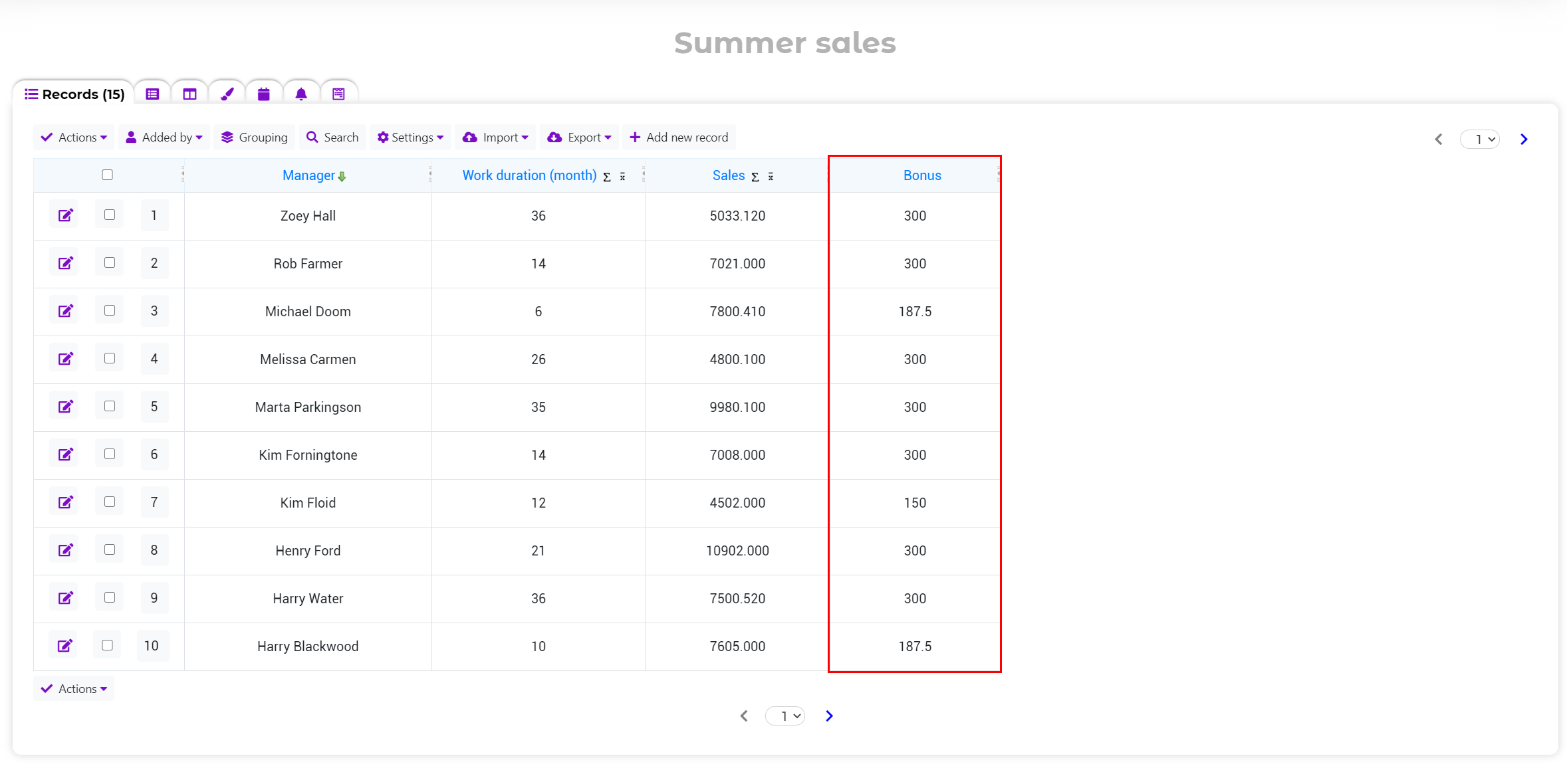Available methods and operators: to_f, to_i, to_s, round, floor, ceil, ceiling_up, ceiling_down, include?, if, elsif, else, end.
To check if the field contains a specific value you can also use [ ] element.

Example usage:

if 'supplies' ['stock']
true
else
false
end

Also, you can use two variables: a and b

In addition to arithmetic operators, you can use basic trigonometric, logarithmic, transcendental functions and roots. Functions must be written as follows: Math::function(Field_name). For example, Math::acos(Field_csIfon). Detailed list of mathematical functions:

Trigonometric Functions

(::cos) Returns the cosine of the given argument.
(::sin) Returns the sine of the given argument.
(::tan) Returns the tangent of the given argument.

Inverse Trigonometric Functions

(::acos) Returns the arc cosine of the given argument.
(::asin) Returns the arc sine of the given argument.
(::atan) Returns the arc tangent of the given argument.
(::atan2) Returns the arg tangent of two given arguments.

Hyperbolic Trigonometric Functions

(::cosh) Returns the hyperbolic cosine of the given argument.
(::sinh) Returns the hyperbolic sine of the given argument.
(::tanh) Returns the hyperbolic tangent of the given argument.

Inverse Hyperbolic Trigonometric Functions

(::acosh) Returns the inverse hyperbolic cosine of the given argument.
(::asinh) Returns the inverse hyperbolic sine of the given argument.
(::atanh) Returns the inverse hyperbolic tangent of the given argument.

Exponentiation and Logarithmic Functions

(::exp) Returns the value of a given value raised to a given power.
(::logReturns the logarithm of a given value in a given base.
(::log10) Returns the base 10 logarithm of the given argument.
(::log2Returns the base 2 logarithm of the given argument.

Fraction and Exponent Functions

(::frexp) Returns the fraction and exponent of the given argument.
(::ldexp) Returns the value for a given fraction and exponent.

Root Functions

(::cbrt) Returns the cube root of the given argument.
(::sqrt) Returns the square root of the given argument.

Error Functions

(::erfReturns the value of the Gauss error function for the given argument.
(::erfc) Returns the value of the complementary error function for the given argument.

Gamma Functions

(::gamma) Returns the value of the gamma function for the given argument.
(::lgamma) Returns the value of the logarithmic gamma function for the given argument.

Hypotenuse Function

(::hypot) Returns sqrt(a**2 + b**2) for the given a and b.

## String functions

You can capitalize the text this way:Make sure to quote your variable and then use 'capitalize' function.

## Tips & Tricks

Here is some examples of how to simplify your work using functions.

We have a Job candidates table: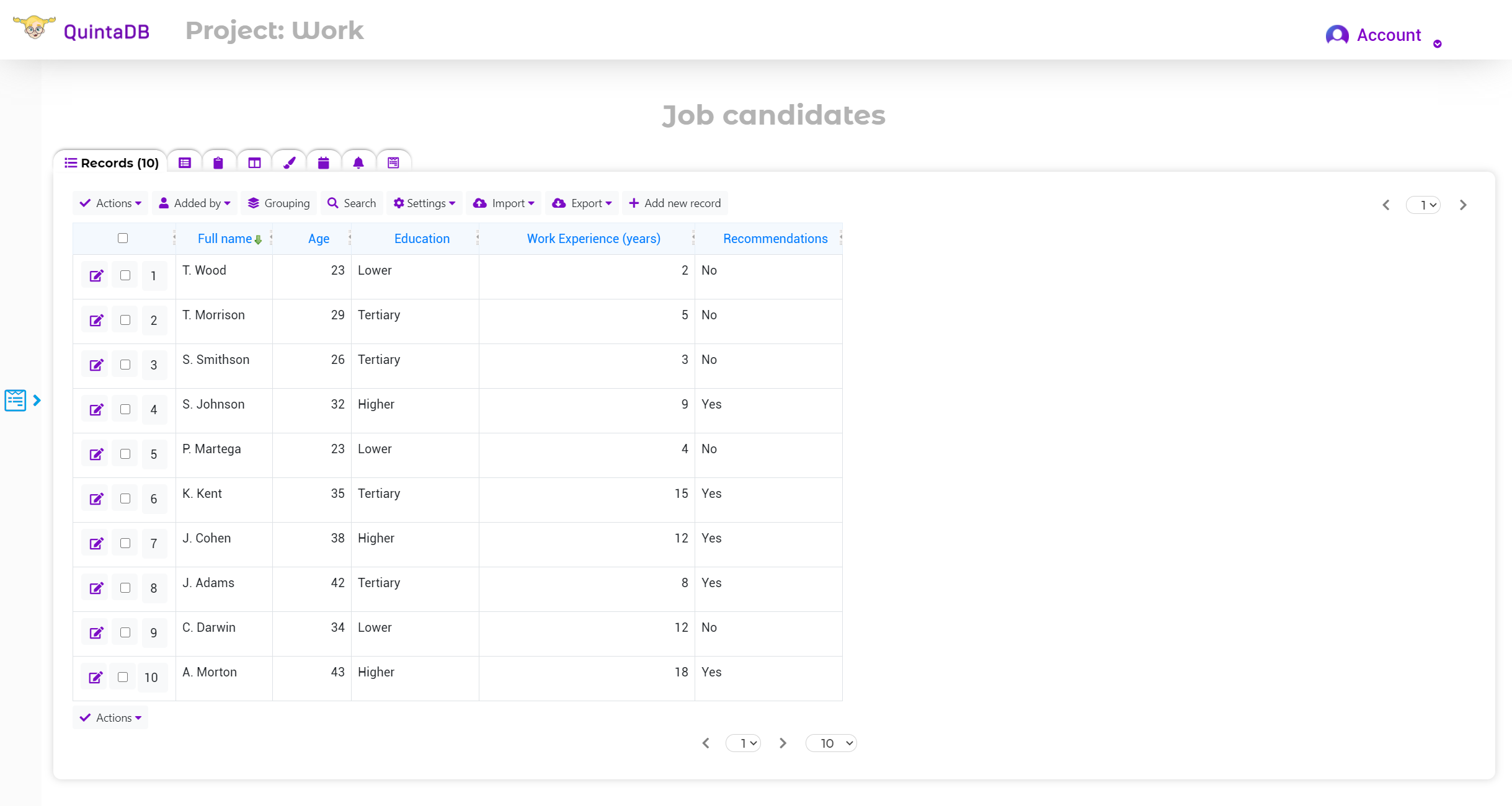The task is to divide candidates into three groups: a suitable candidate, a reserve candidate and an unsuitable candidate.

We can use the Search Form, but then we'll have to use all criteria: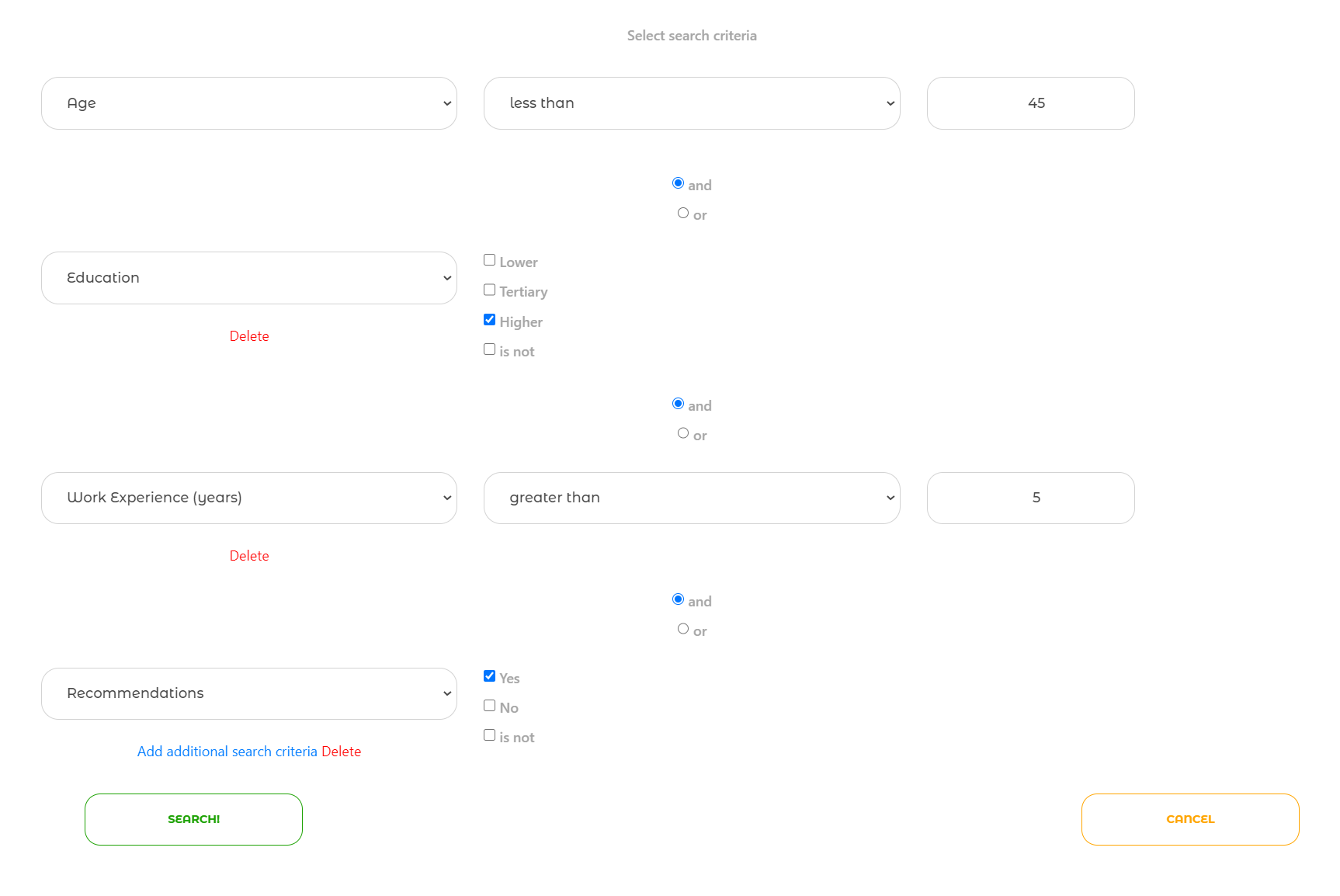A Formula field with a function comes to the rescue. Let's create a new "Selection" formula.

We've to divide the candidates into three conditional groups.

If the candidate has:

- Age less than 45 years old;

- Higher education;

- Work experience over 5 years;

- A recommendation from a previous employer, then such a candidate receives the "Suitable" status.

For the "Reserve" group a candidate needs less than 50 years old, higher or tertiary education and more than 5 years of work experience.

All other candidates receive the status "Unsuitable ".

Let's write the following code for the function:

if age<45 && "education" == "Higher" && exp>5 && "recommend" == "Yes"
"Suitable"
elsif age<50 && "education" == "Higher" || "Tertiary" && exp>5
"Reserve"
else
"Unsuitable "
end

Please note: numeric values (including html field names) are not quoted. Quotes are used for string values only.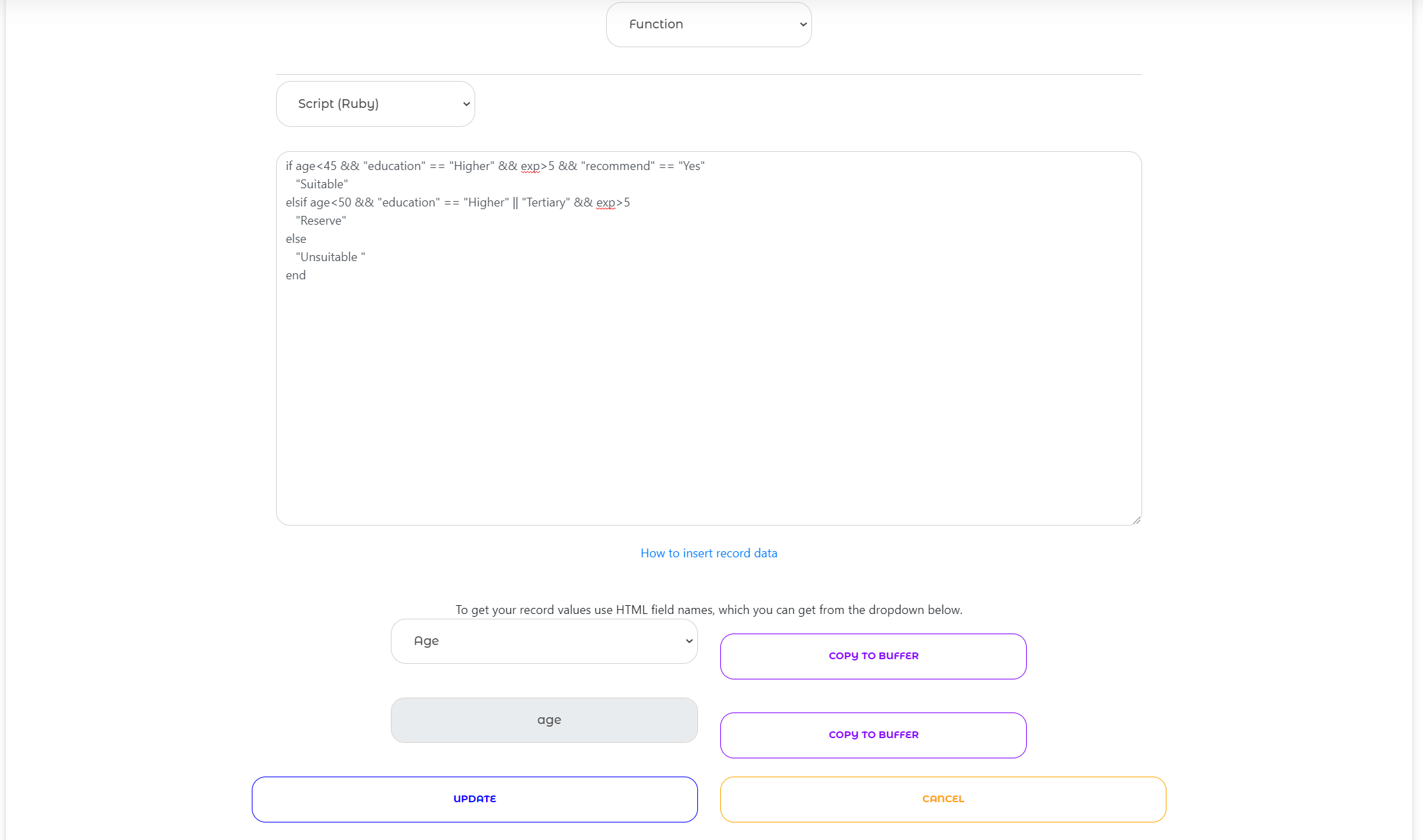As you can see in the following screenshot, each candidate was assigned a status based on the criteria: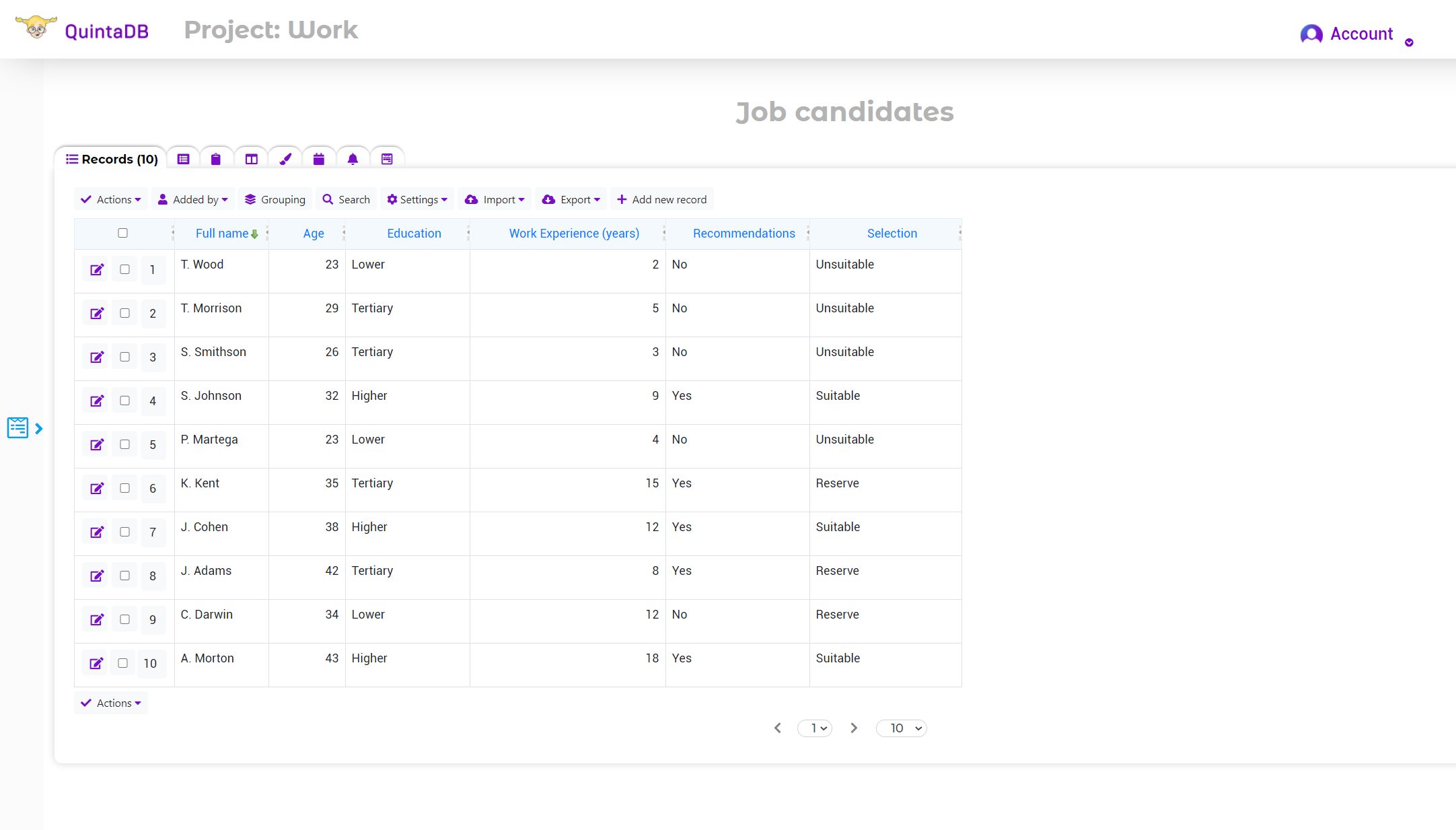It is much easier to search on a single field instead of multiple criteria: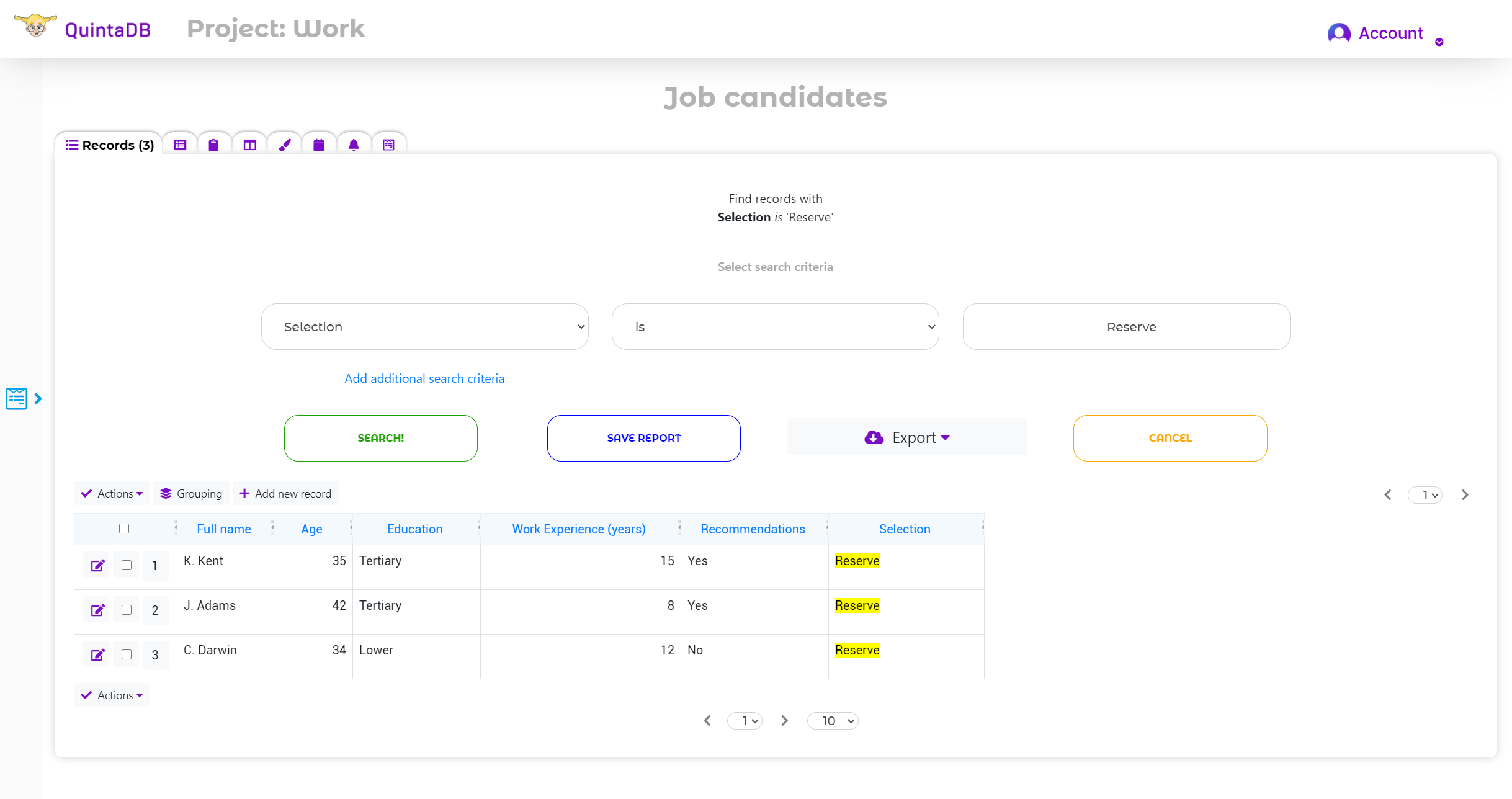The same with Conditional formatting in a table: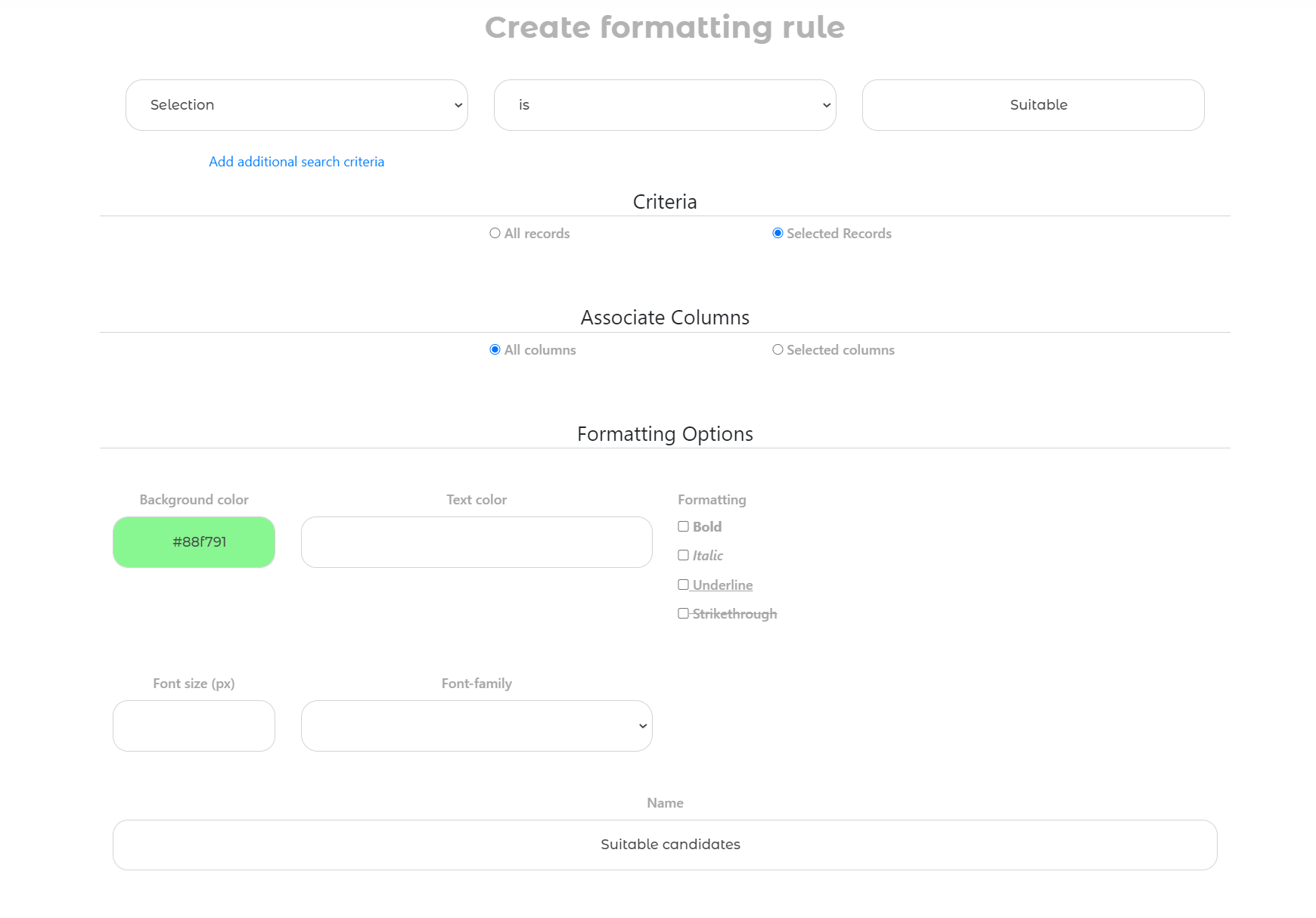We use the "Selection" field to format records in the table: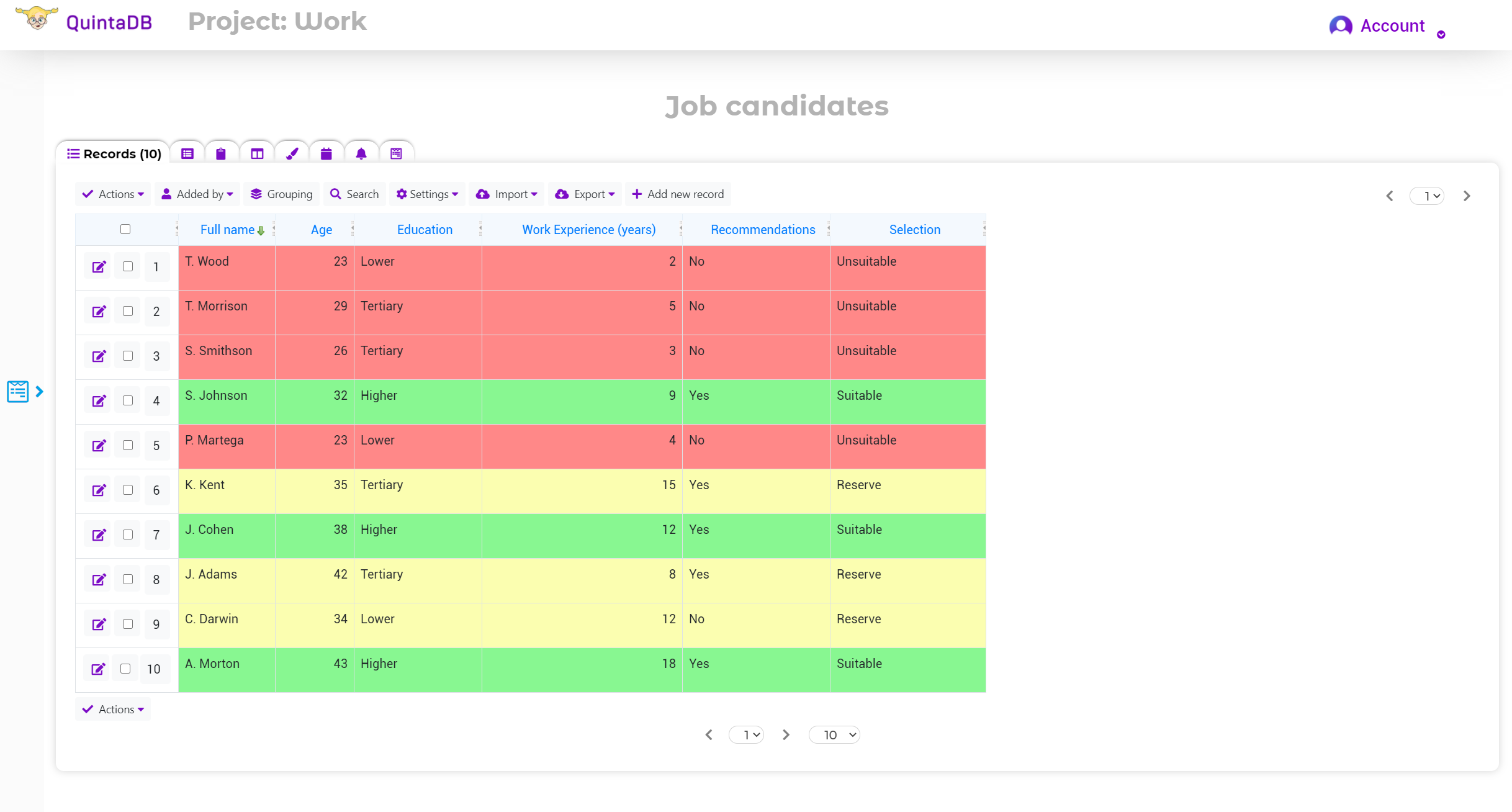It is much more convenient to generate a Report after searching or grouping by one field: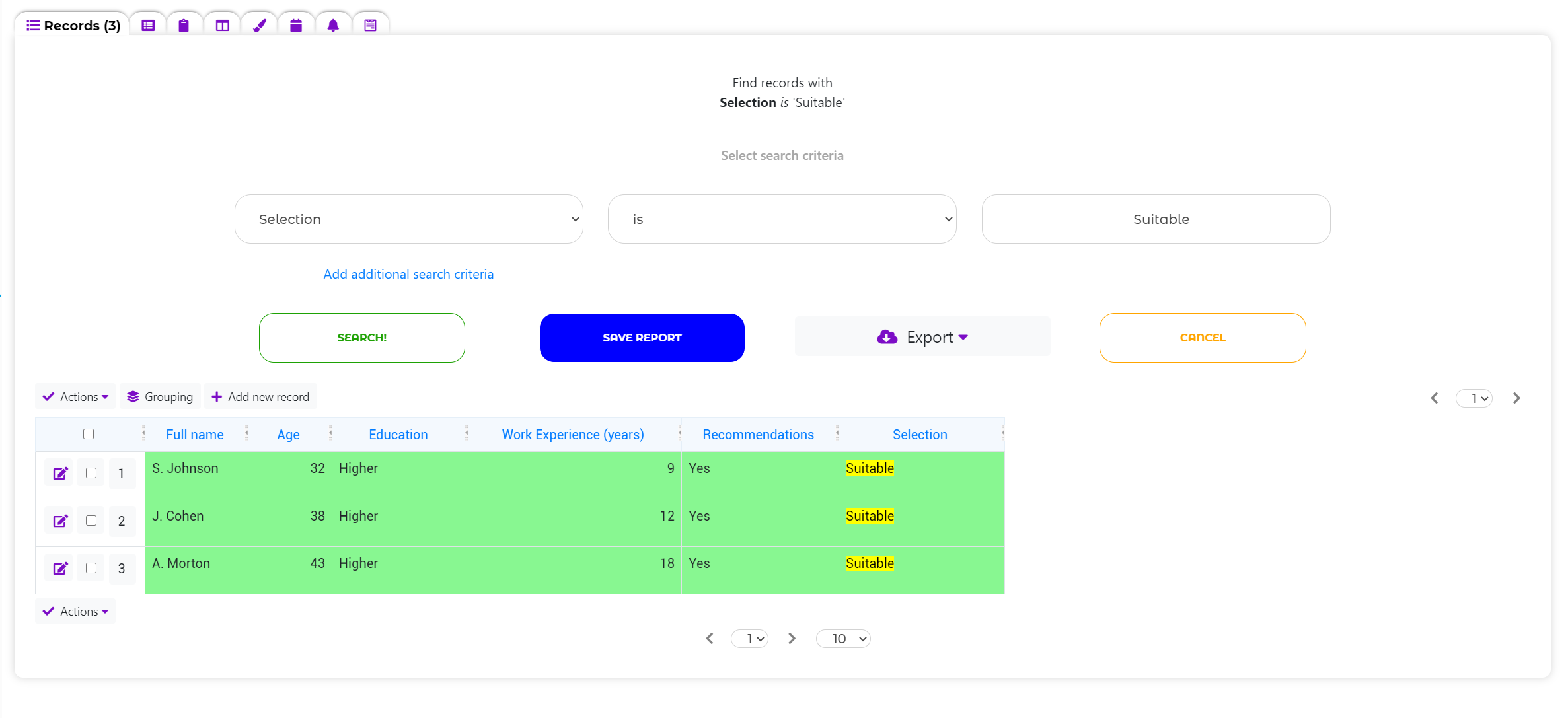Which contains all candidates by selected criteria: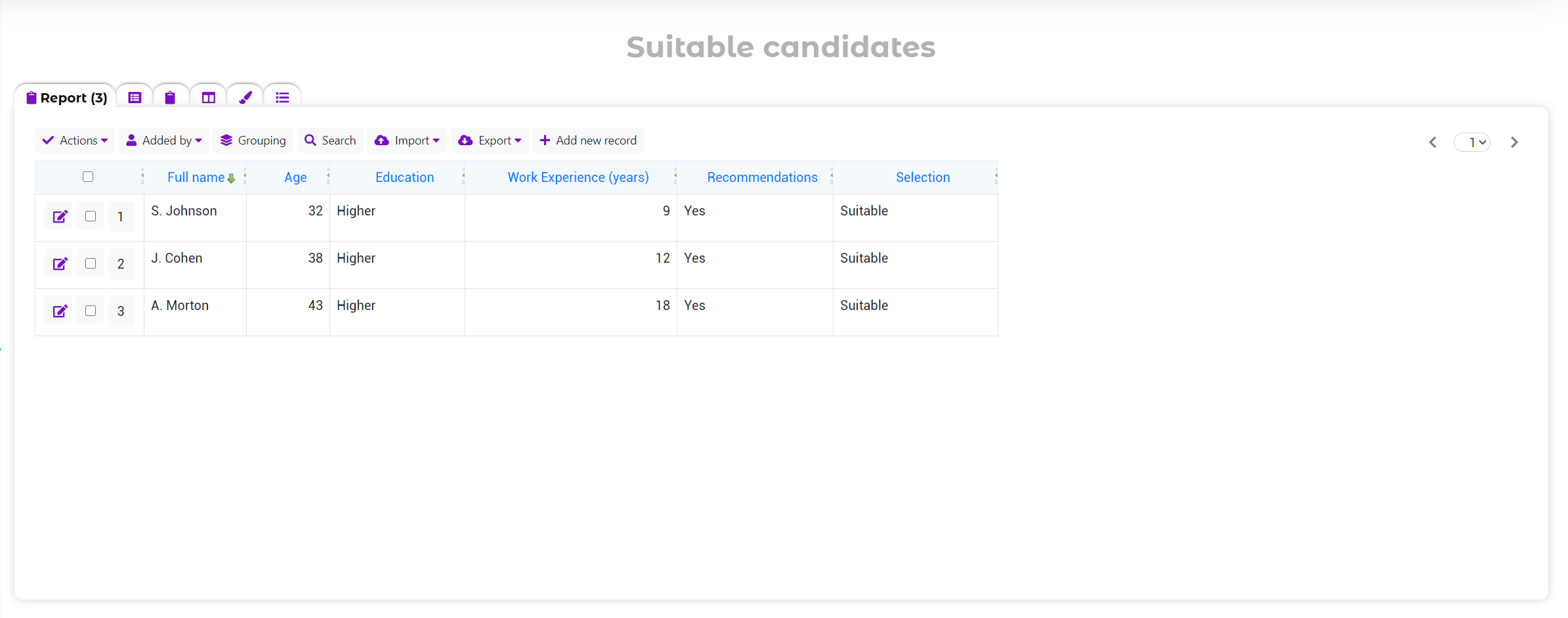Don't forget about Conditional Actions and the Action field.

For example, create a button with the action "Send Email", which is available in the record with the "Suitable" candidates only.

You can see an example of how to create an Action button by this link.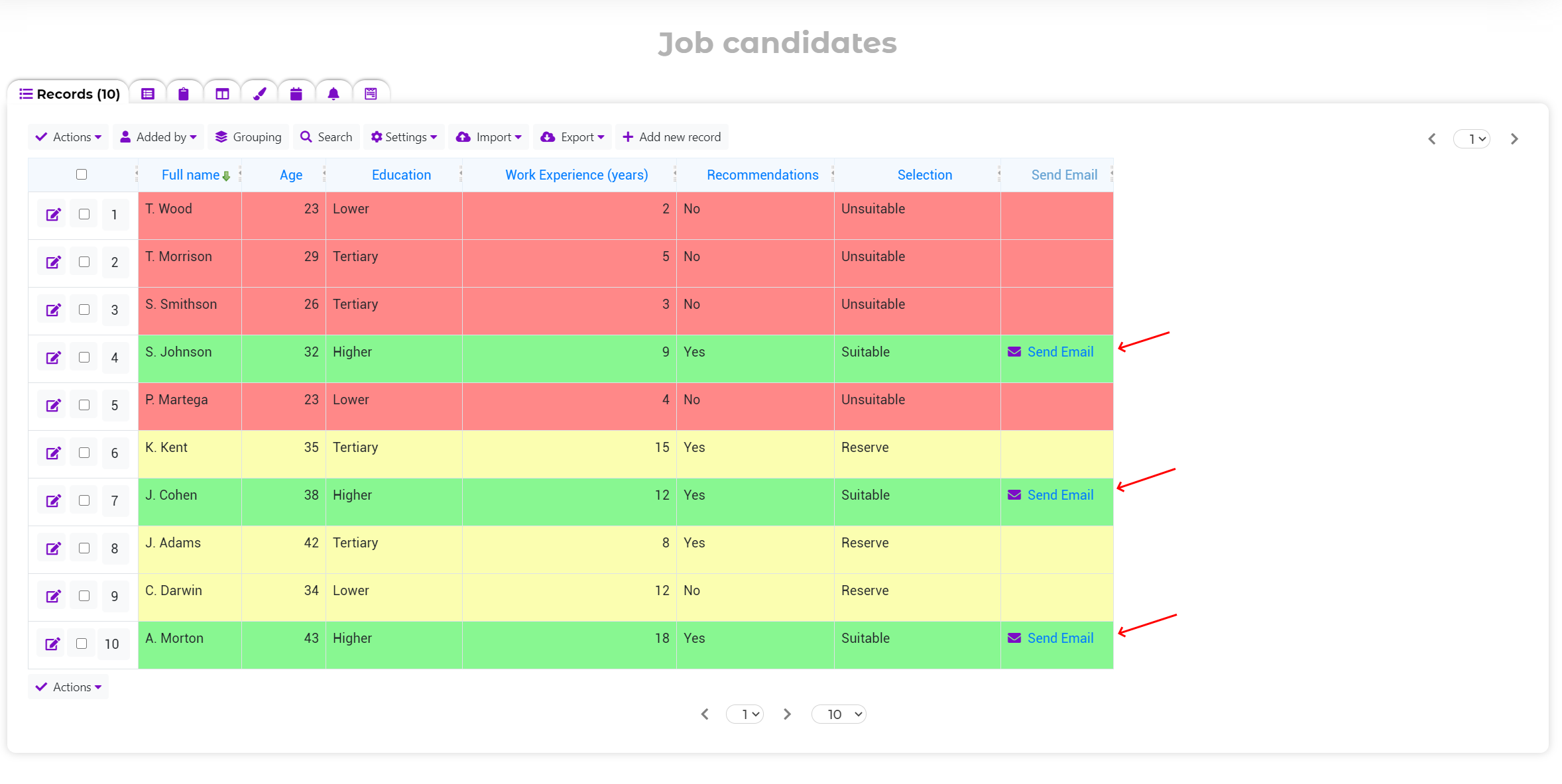A function field can be used as a criterion in Field Rules.

As an example, enable the "Selection" field on the form: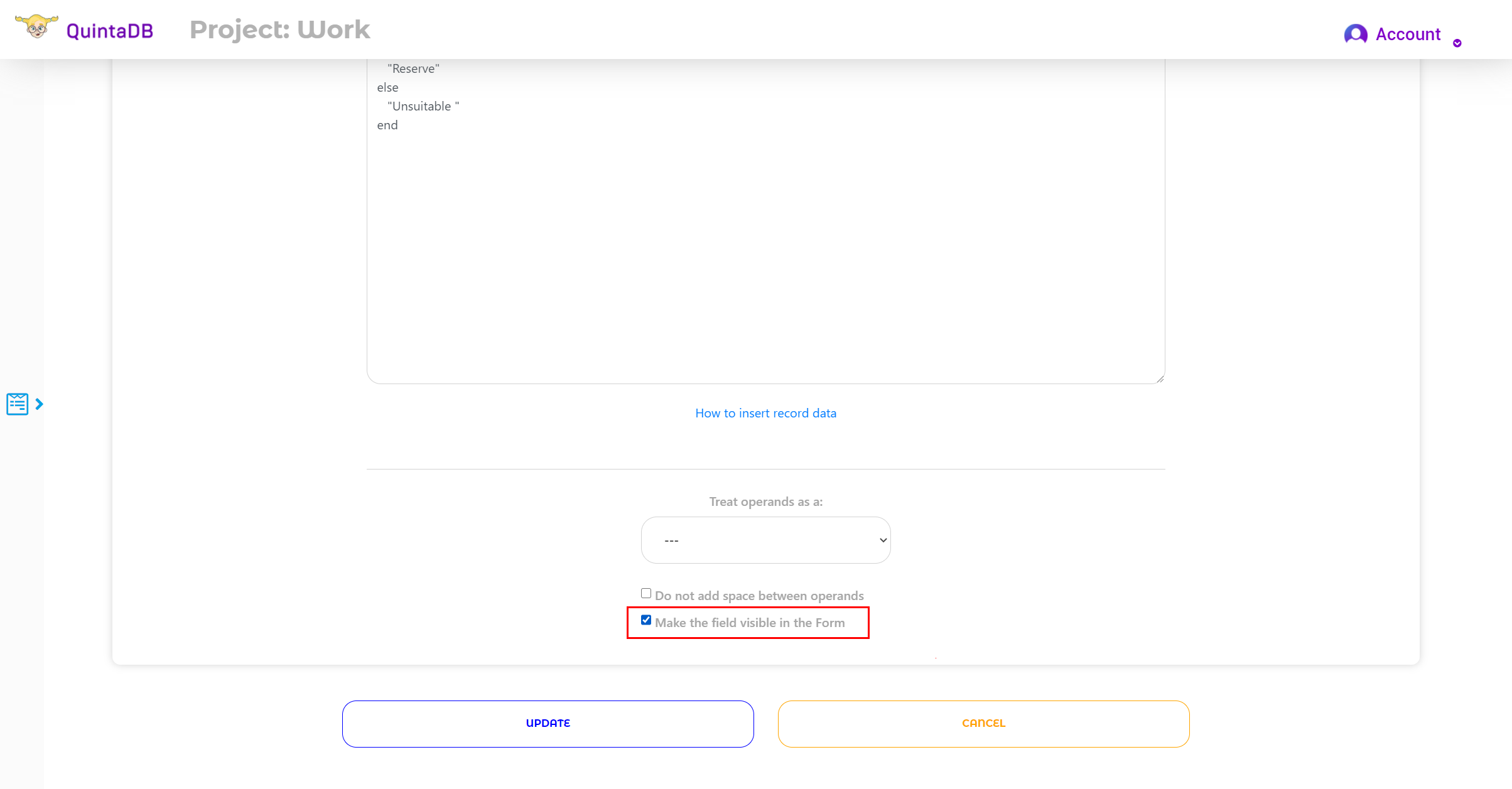Next, let's create a new field "Add to queue" (Radio button) with a choice of two values: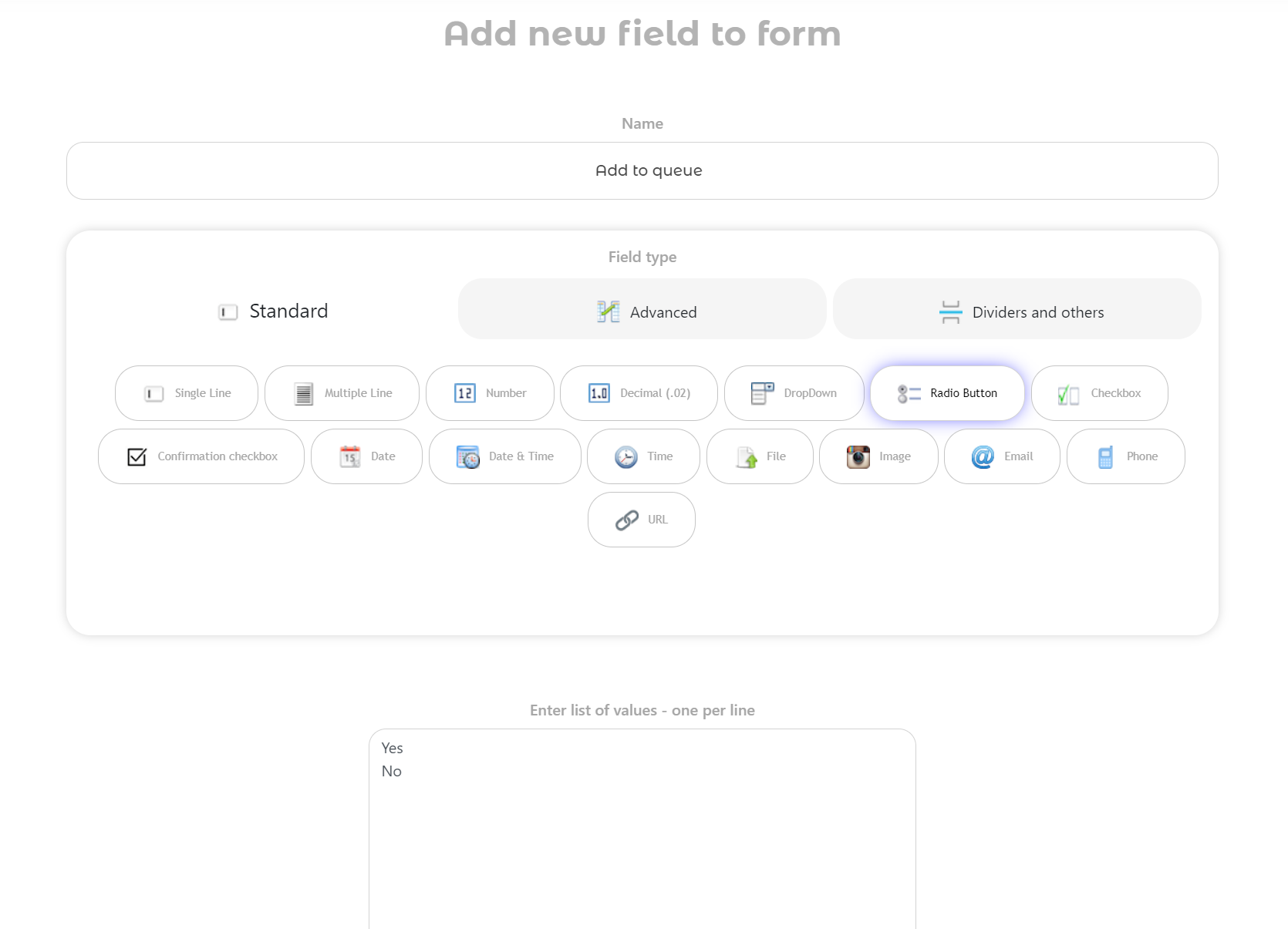Create a rule.

If the "Selection" field consists "Reserve", then show the "Add to queue" field: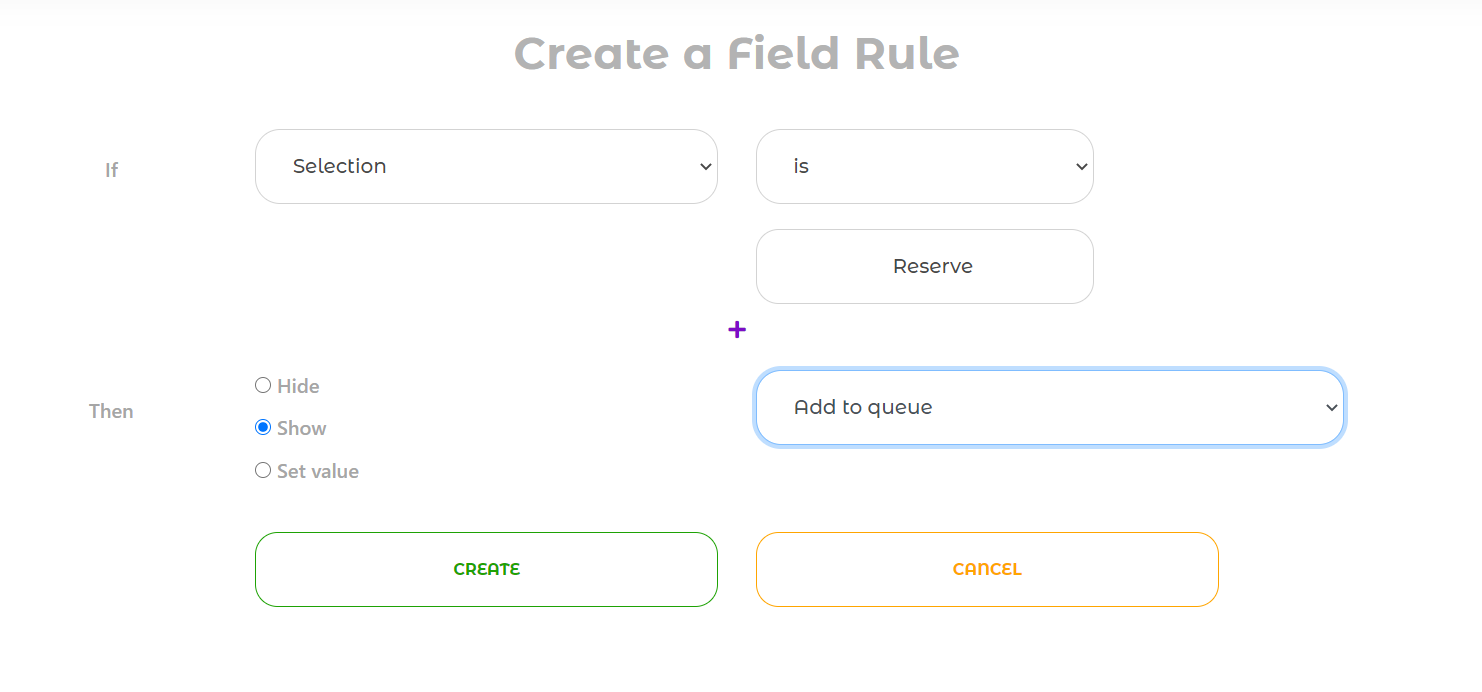This is how it looks on the form:The functions will allow not only more efficient data management, but also more complex calculations.

For example, consider a Profit definition table: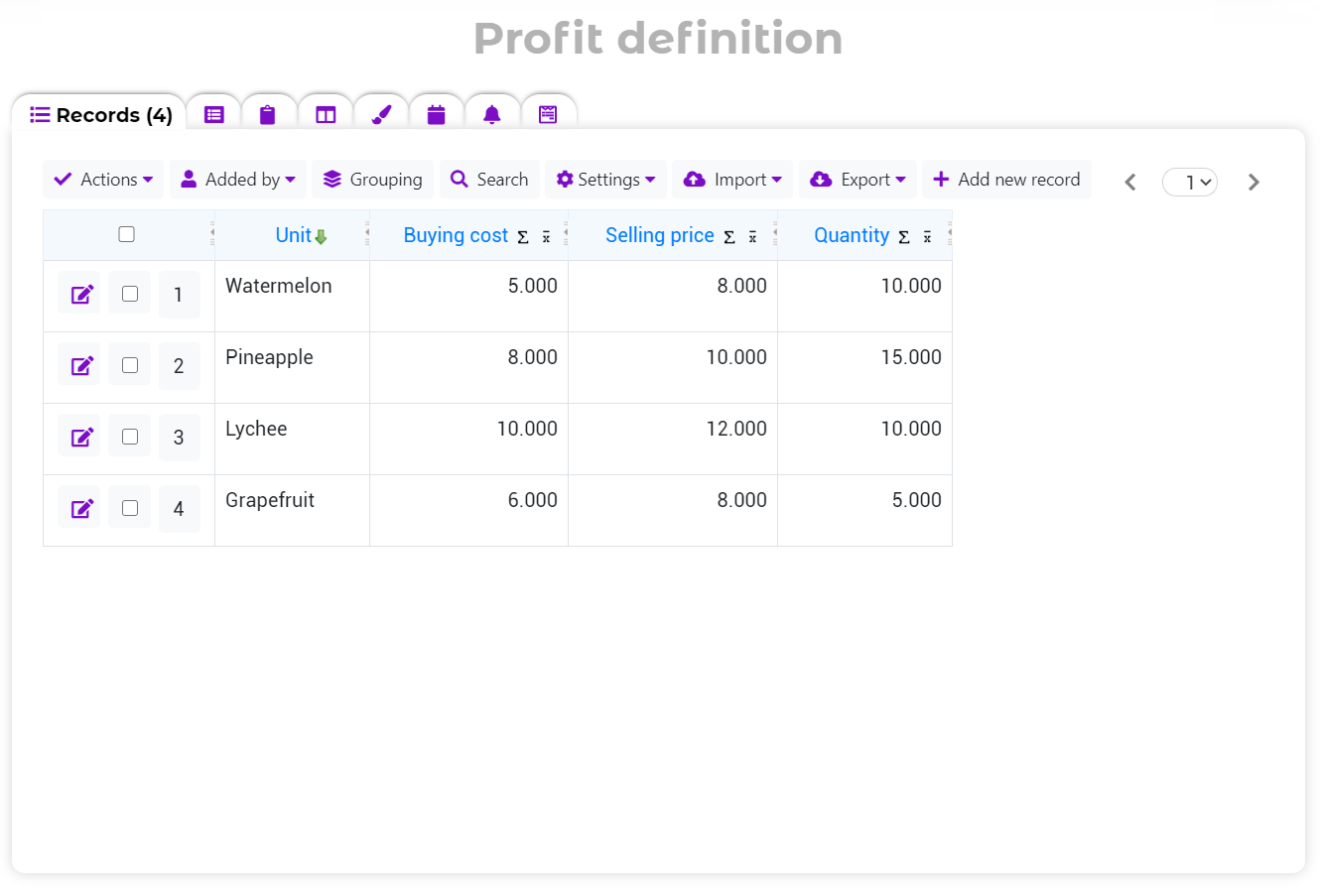The task is to calculate total profit.

The formula looks like this: total profit = revenue - total cost

If we carry out such a calculation using standard formulas, then we would have to divide the calculation into several formulas (separately calculate revenue and total cost).

Using Ruby functions, you can do this calculation in one field: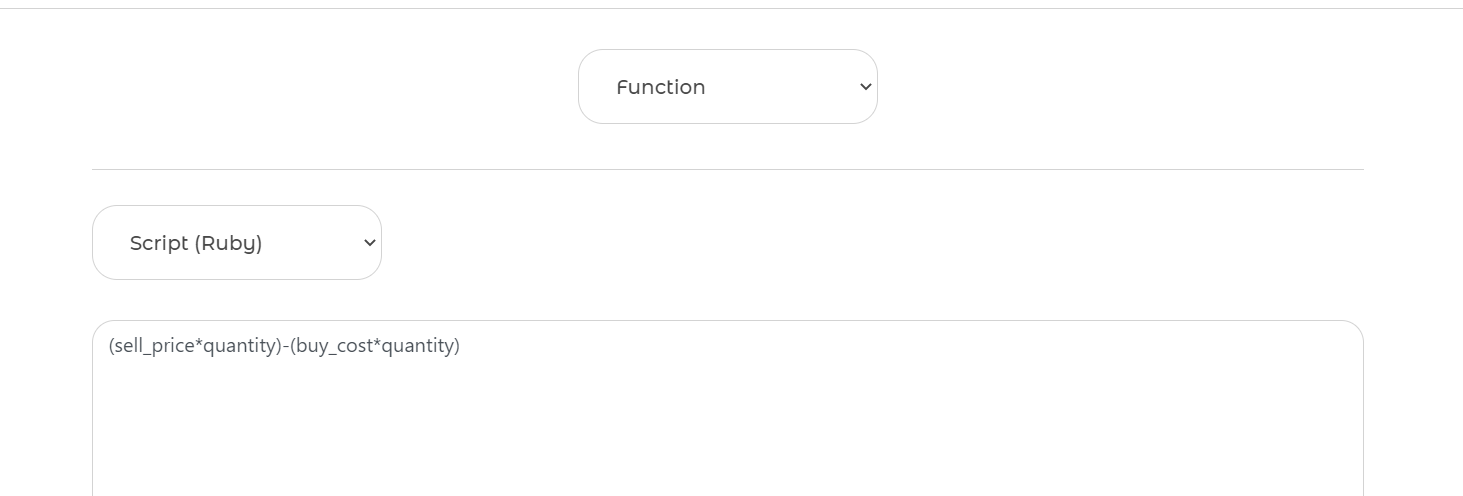Total profit in table: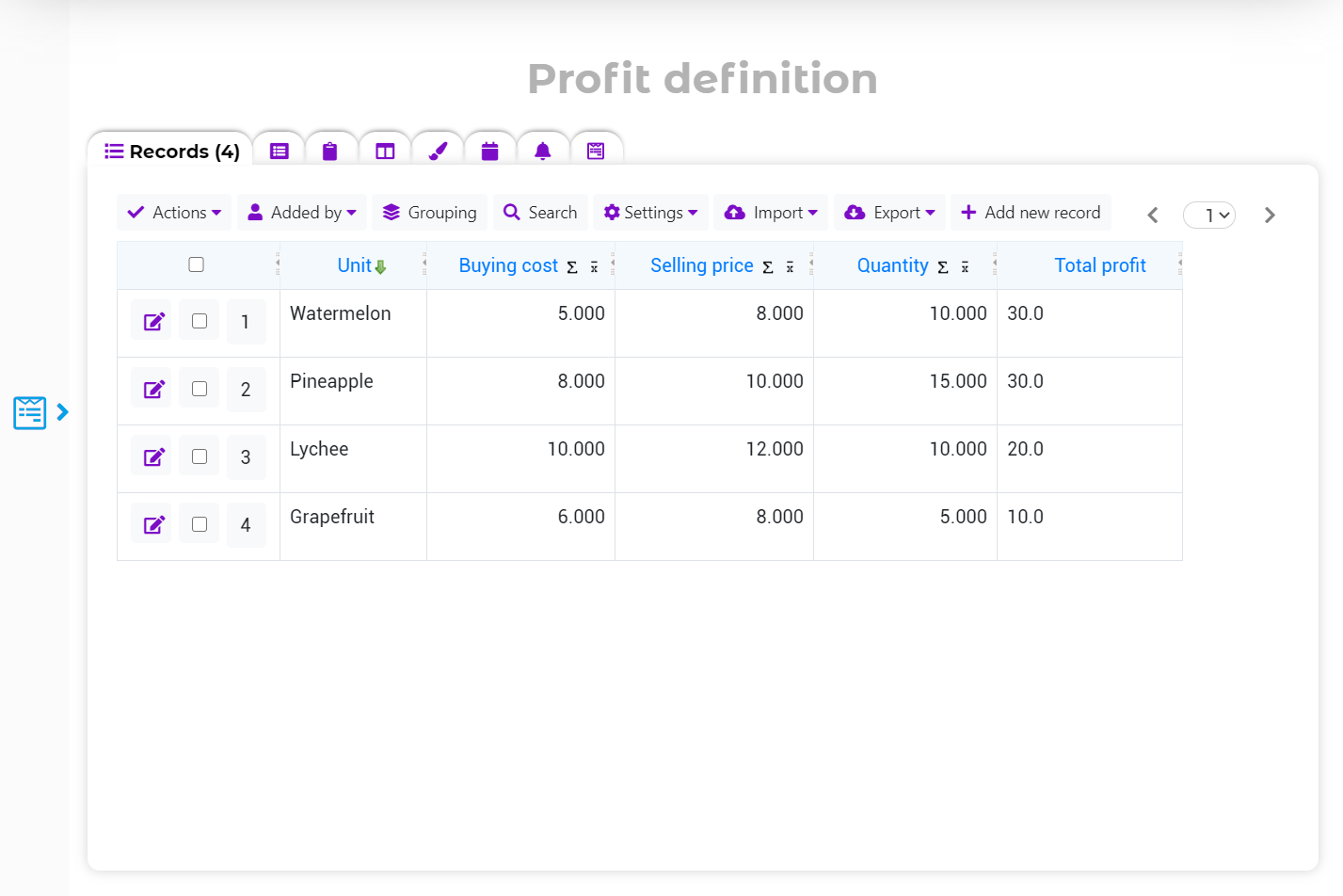Please note: for correct calculation, you must use the "Decimal" field type.

Counting days between dates

For example, we'll use a table with contracts.

Task: show how many days the contract will end.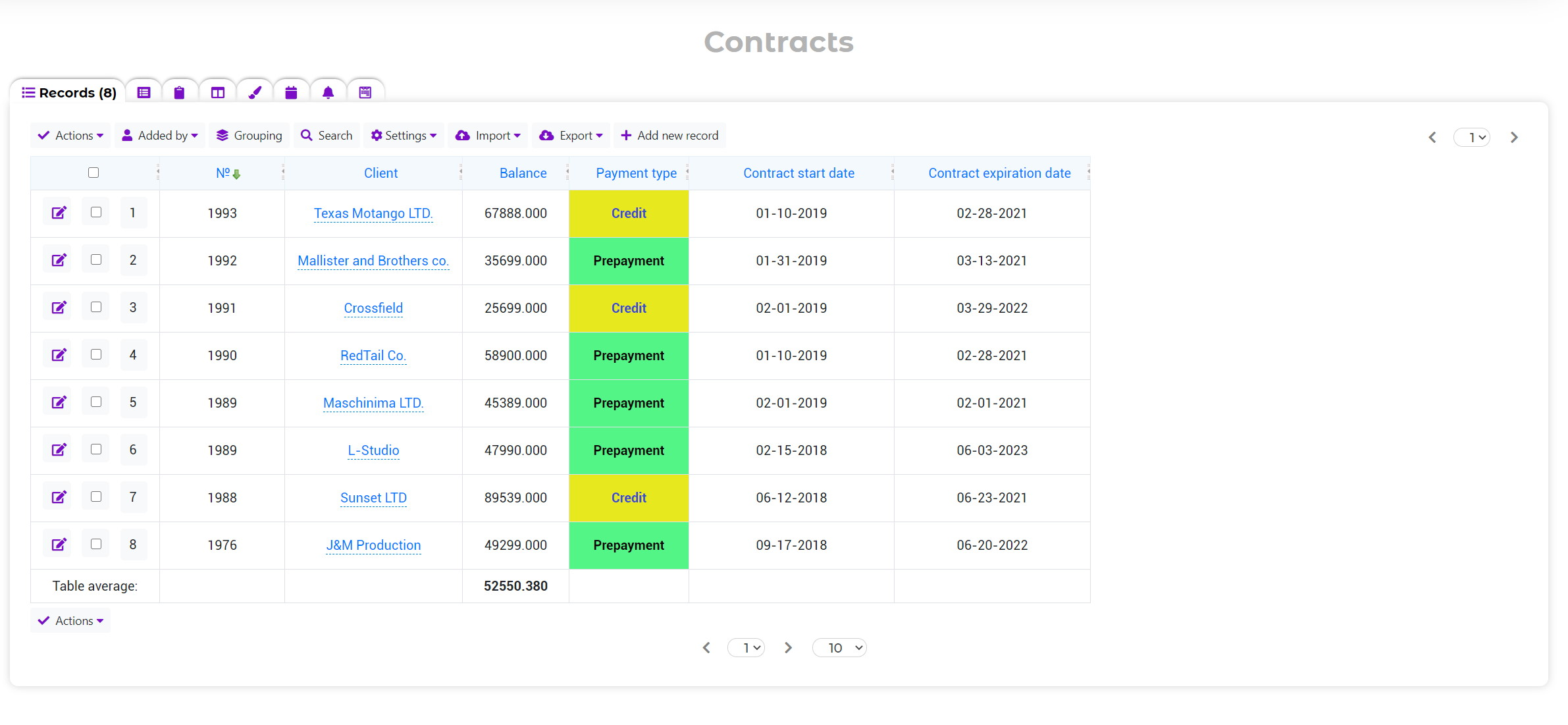Create the following script:

exp_date.mjd - DateTime.now

Where:

exp_date - html field name.

.mjd method - converts the date to the Julian calendar format.

DateTime.now - object whose value is the current local date and time.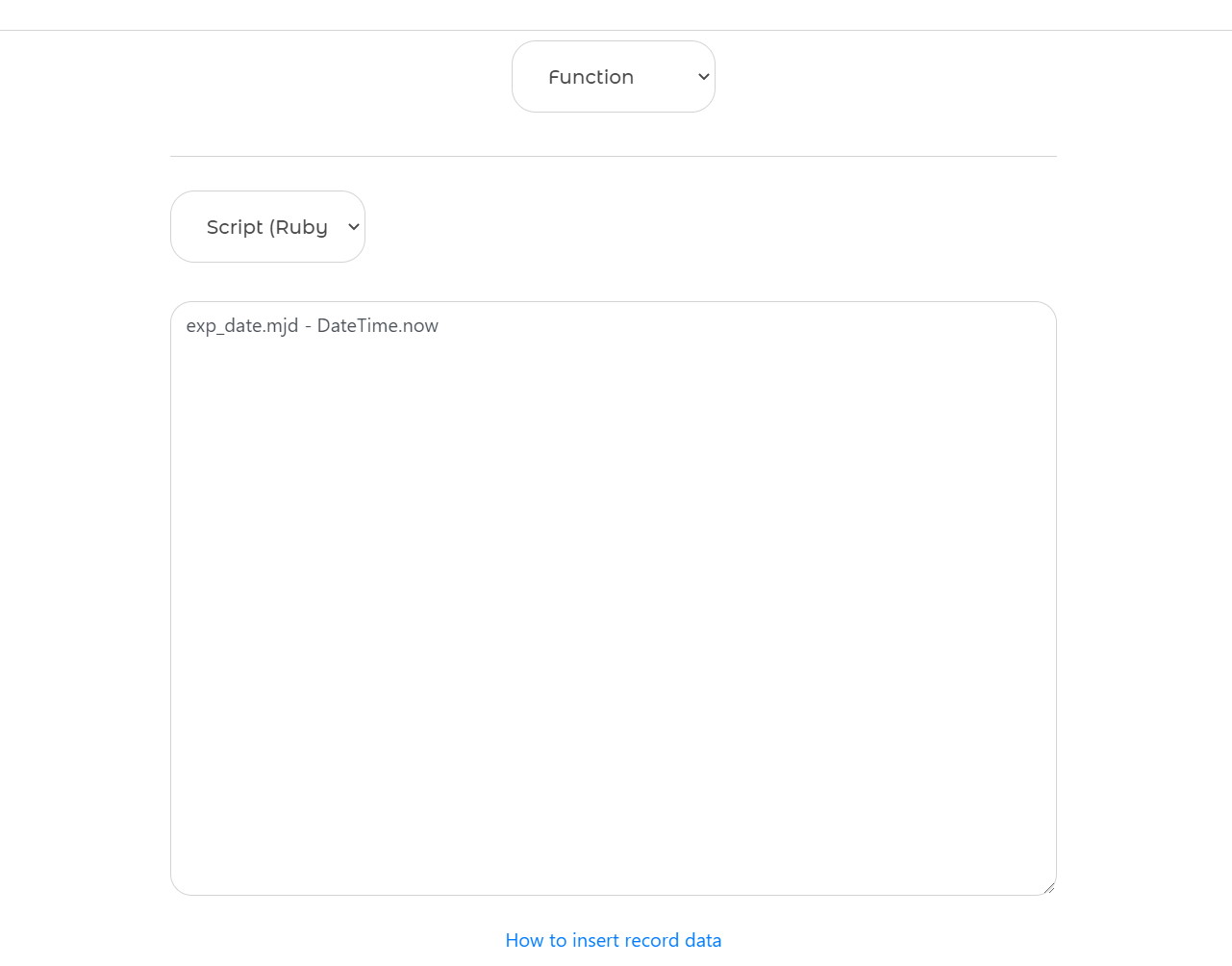As a result, we get a column with the number of days until the end of the contract.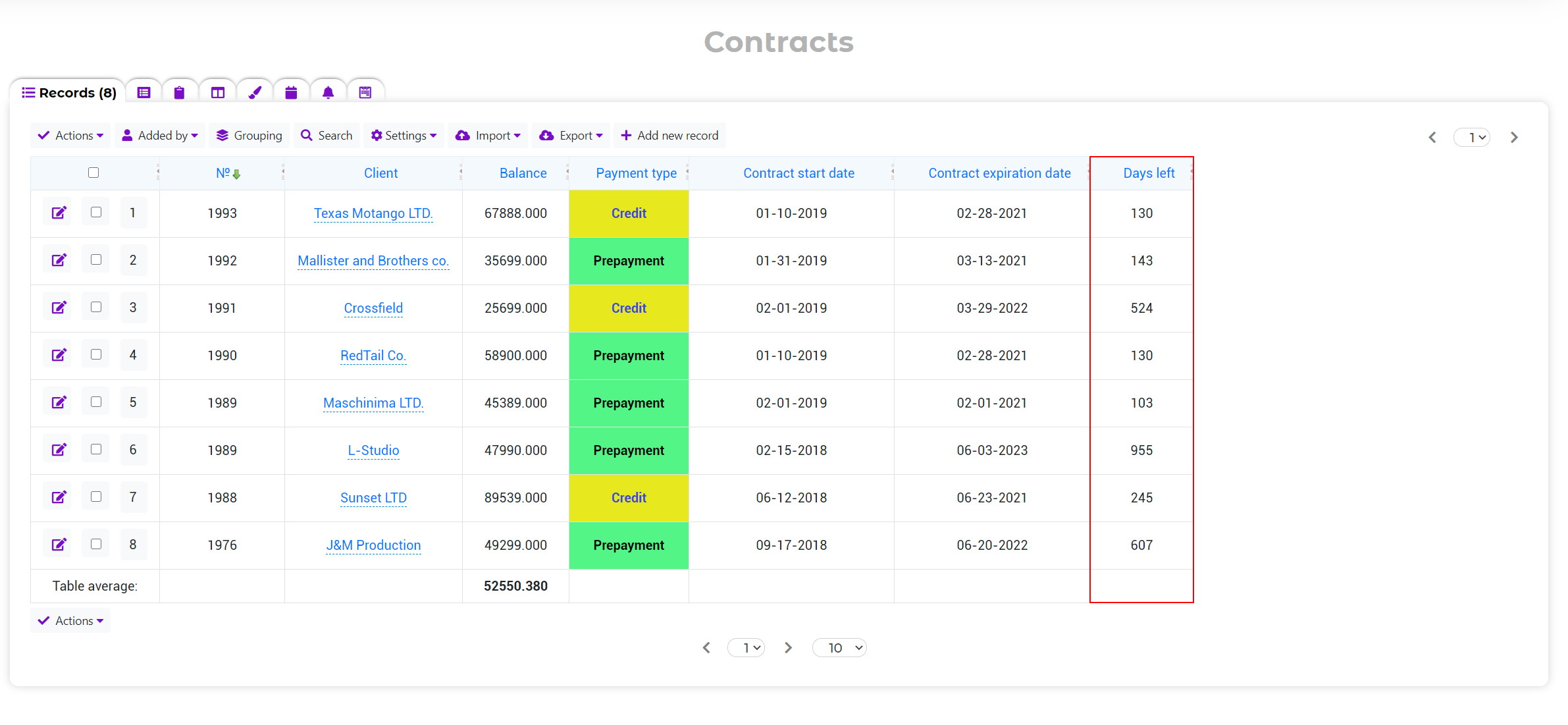Here is another example.

Task: determine the year of birth, having only the age of a person.

Create the following script:

DateTime.year - age

Where:

age - html field name.

DateTime.year - object whose value is the current year.As a result, we get a column with the year of birth for each person.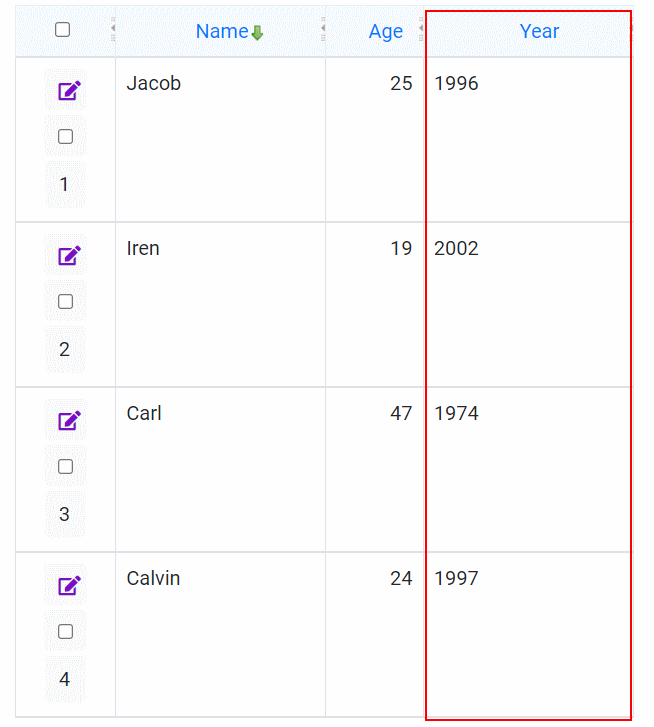Using the script, you can create a link to the record view. Go to the "Records" menu and open the record view.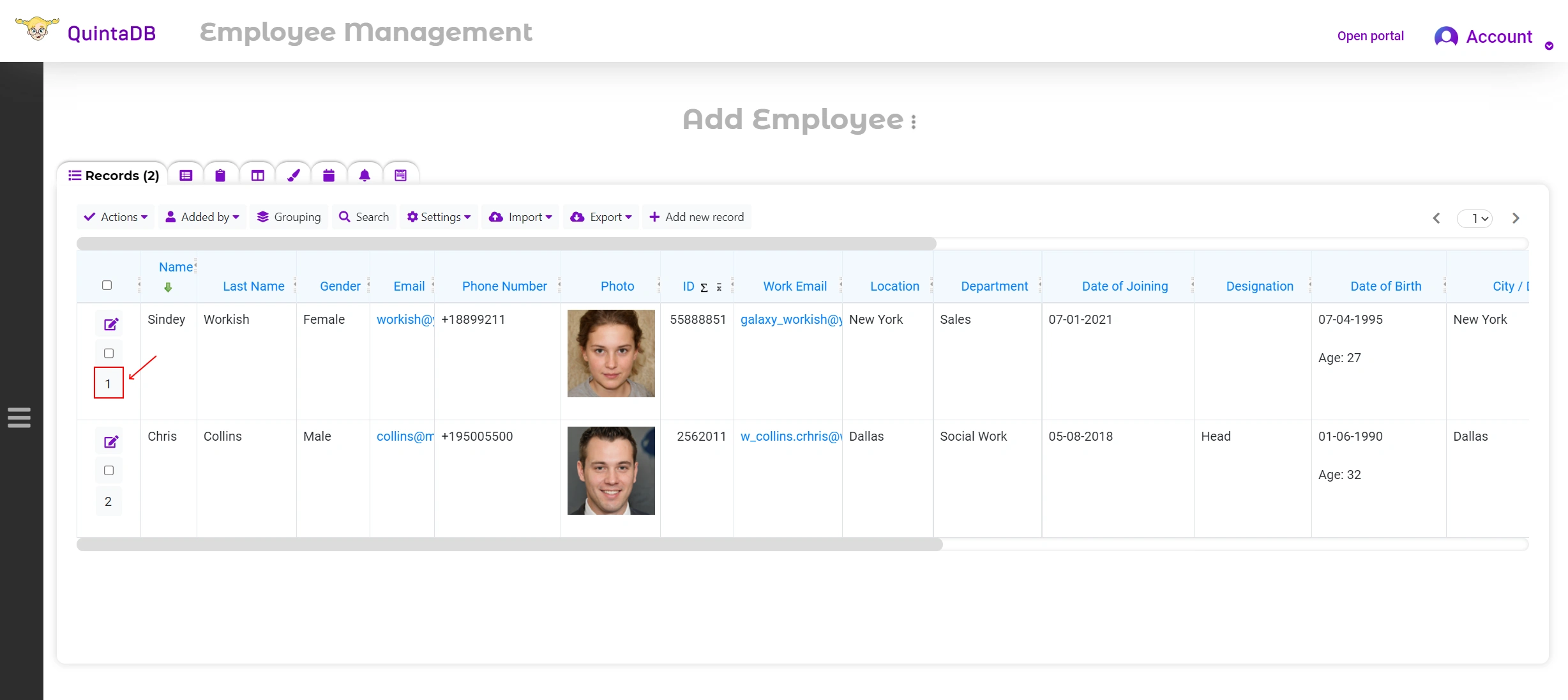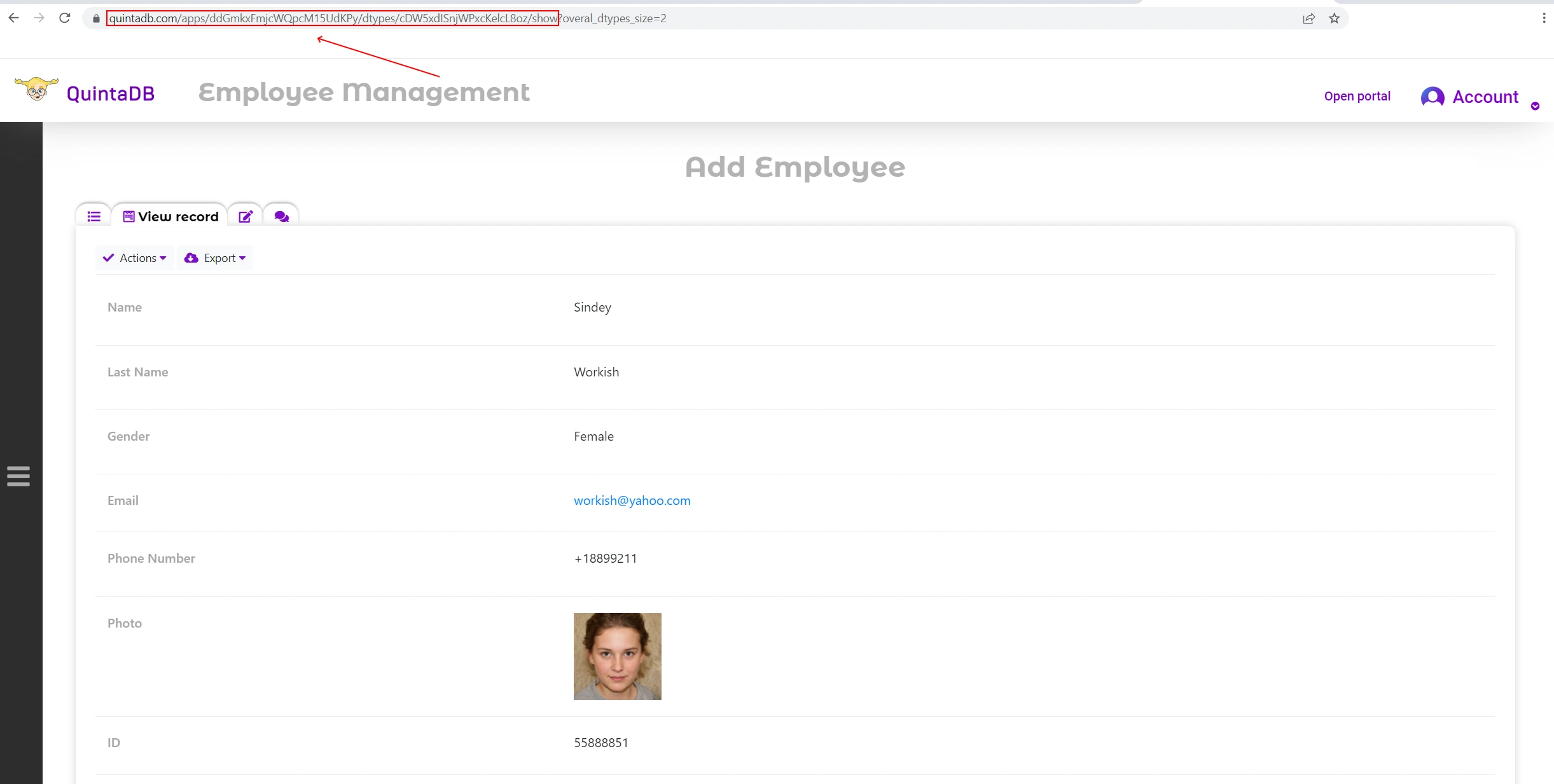Create a Formula field. Select "Script (Ruby)" from the list. You need to use the variable "a" and a record link from the previous step (written in quotes). Replace record id with "Entry ID number" from the list by |internal_id| value: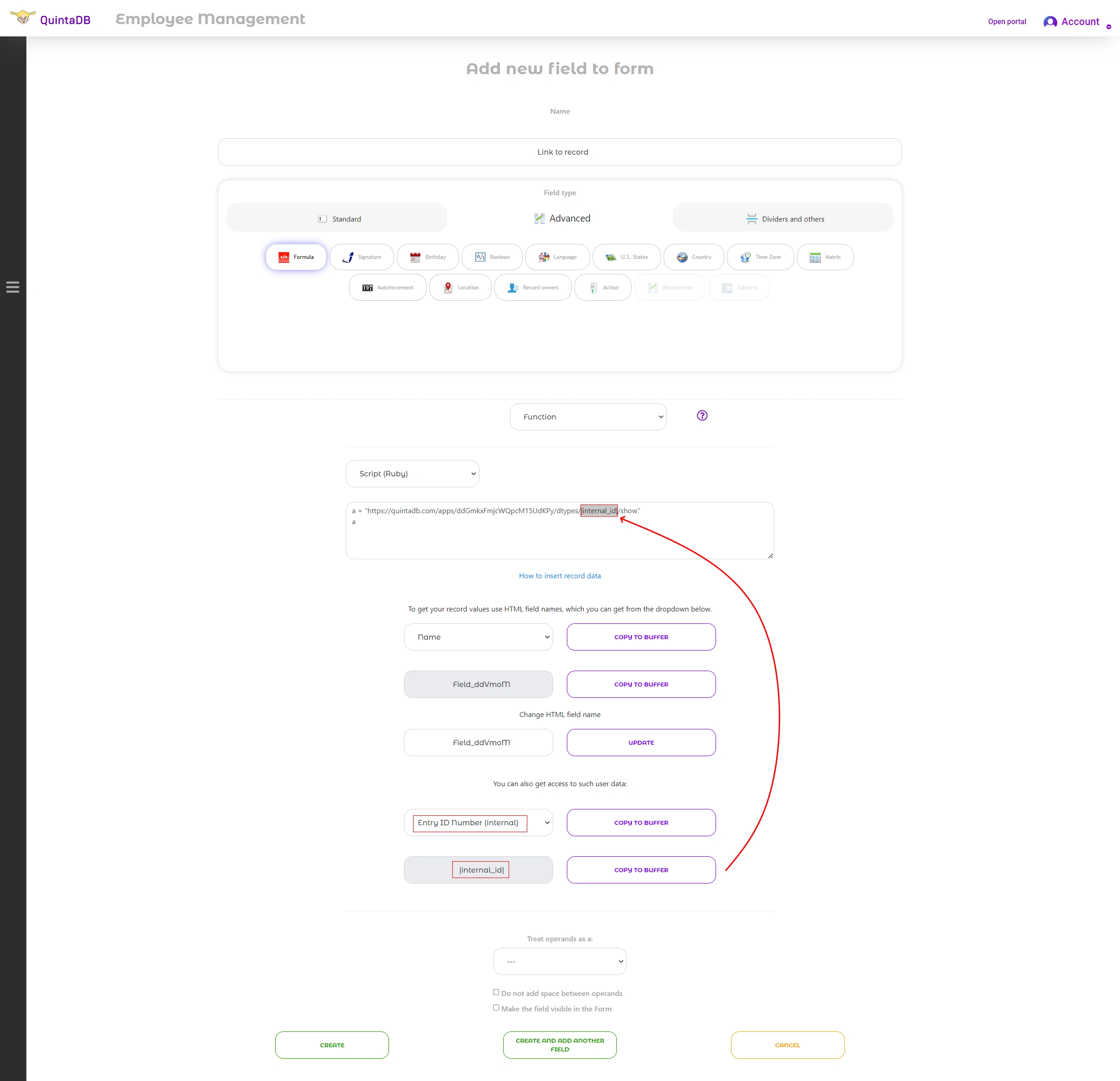Thus, you will get a link to the record in the table: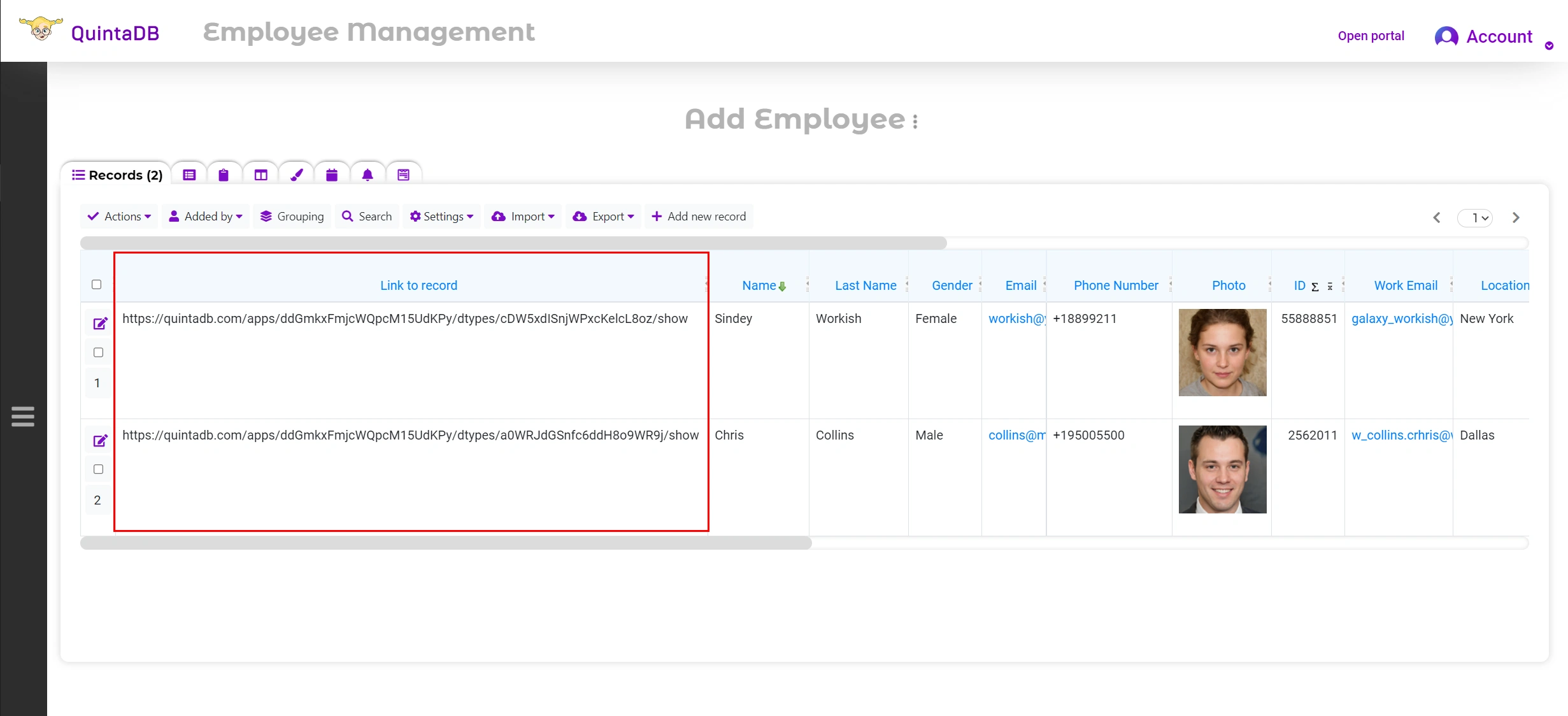You can use a link to the Record widget in the same way:News

Examples

Articles

All posts